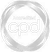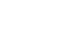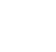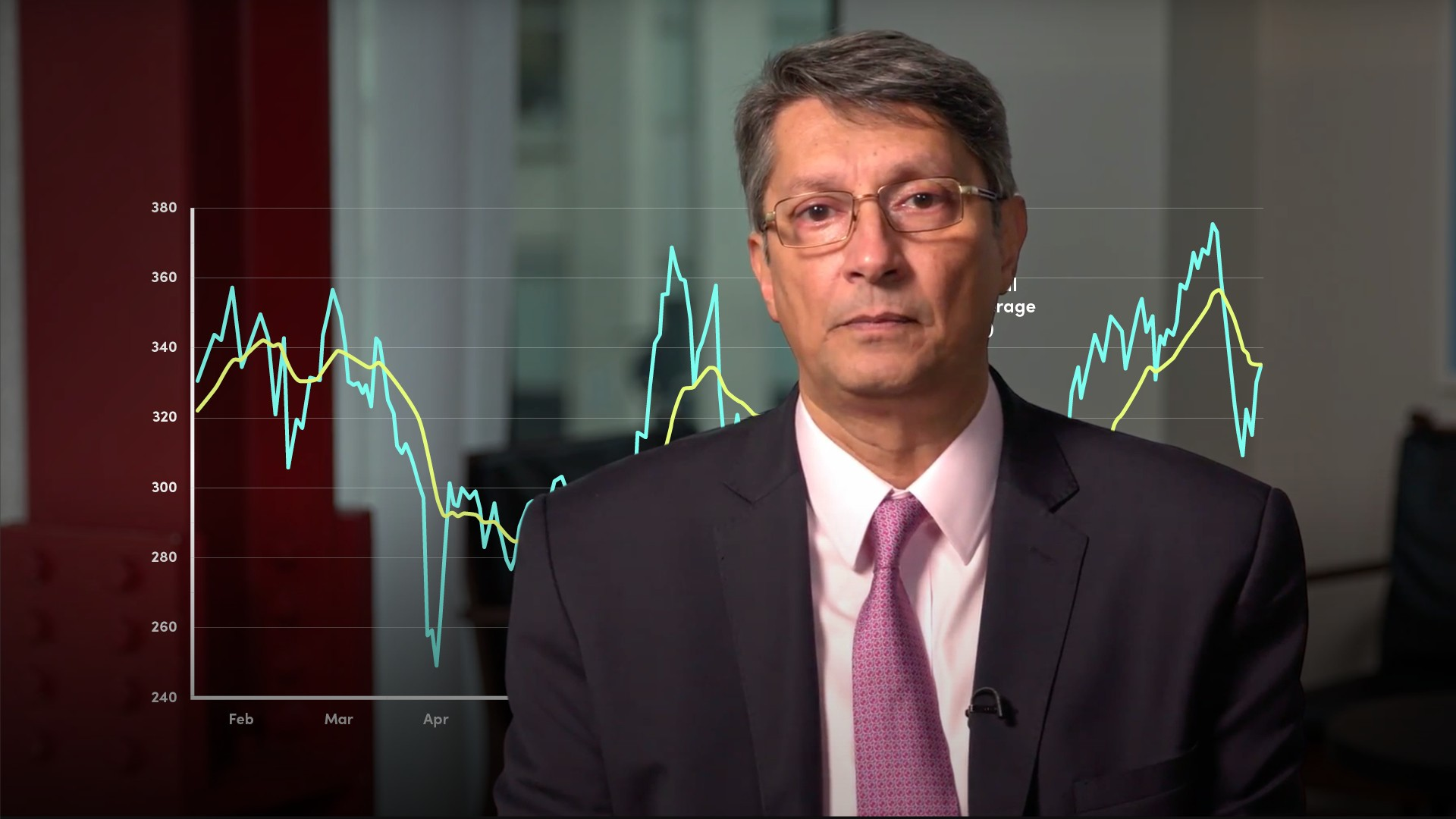# Exponentially Weighted Moving Averages### Abdulla Javeri

In the previous video, Abdulla covered simple or arithmetic moving averages. Here, Abdulla explains a similar concept: the weighted and exponential moving averages. He answers the questions: what are they, how are they calculated and how are they used?

Financial MathsIn the previous video, Abdulla covered simple or arithmetic moving averages. Here, Abdulla explains a similar concept: the weighted and exponential moving averages. He answers the questions: what are they, how are they calculated and how are they used?

Finance Unlocked is the video learning platform built for finance professionals.### Exponentially Weighted Moving Averages

5 mins 11 secs

Overview

The exponentially weighted moving average places differing weights on historic data. The rate at which the weights exponentially decrease is defined as the decay rate.

Key learning objectives:

• Understand the difference between a simple moving average and an exponentially weighted moving average

• Define the impact the decay rate might have on the output calculation

## Book a demo to access

Summary

#### What is the difference between a simple moving average and an exponentially weighted moving average?

The fundamental difference between a simple moving average and a weighted moving average is that in the latter each data point is given its own weight so the newest data has a greater weight than the oldest data. It’s a way of saying that recent moves are more relevant than older ones. This makes sense especially as various averages are used to forecast future returns.

We often hear end of year ‘forecasts’ for equity indices. The pension industry uses forecasts of future returns to calculate expected levels of pensions. It could be argued that these aren’t actually ‘forecasts’, rather they are a central expectation of future returns, which usually turn out to be inaccurate.

#### How does the exponentially weighted moving average change over time?

There is a specific type of moving average that’s normally used in the markets and that’s the exponentially weighted moving average, EWMA.  In an exponentially weighted moving average, the weights decay smoothly, exponentially.

## Book a demo to access

Expert### Abdulla Javeri

Abdulla’s career in the financial markets started in 1990 when he entered the trading floor of the London International Financial Futures Exchange, LIFFE, and qualified as a pit trader in equity and equity index options. In 1996, Abdulla became a trainer for regulatory qualifications and then for non-exam courses, primarily covering all major financial products.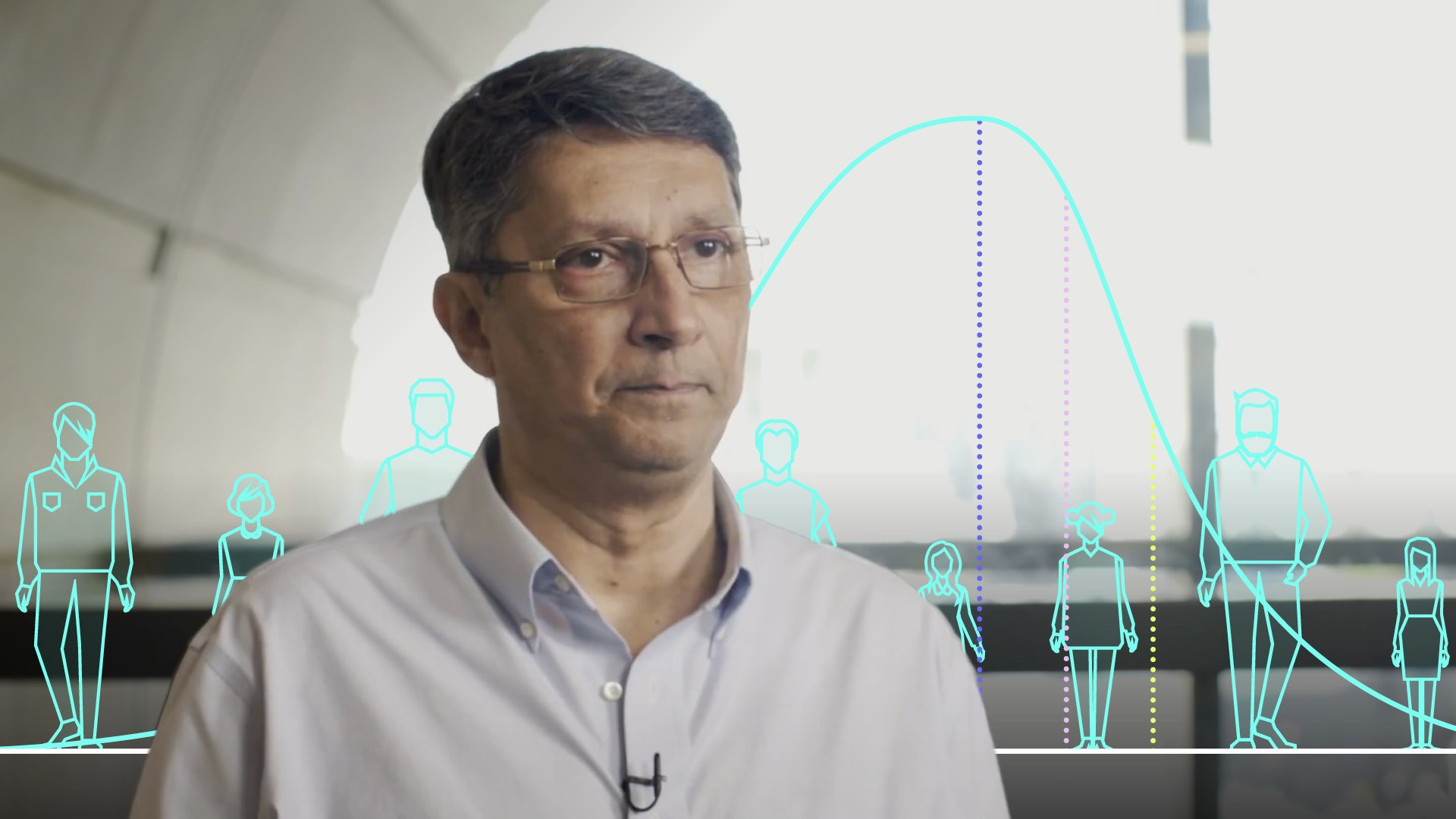03:18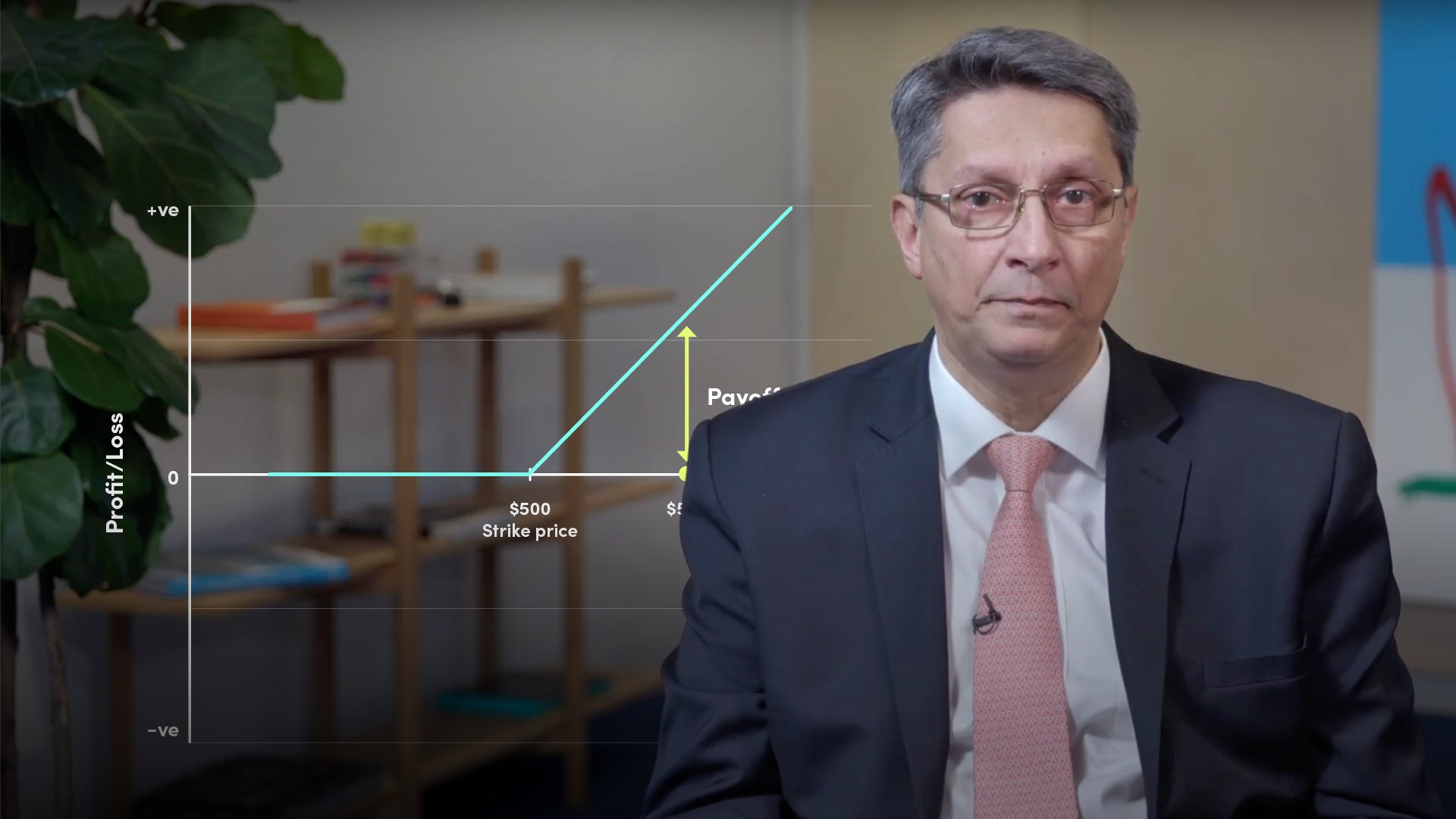05:12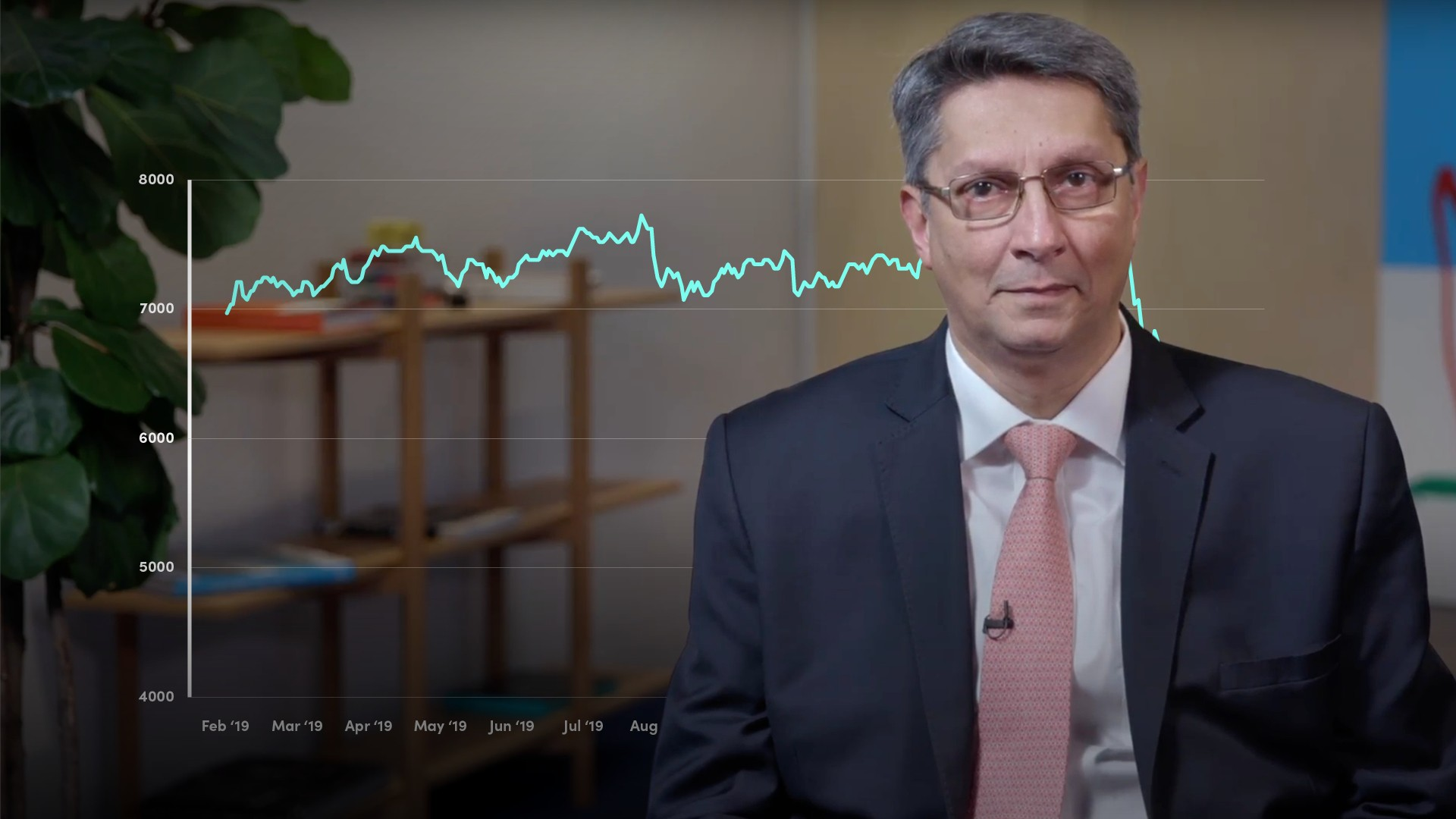05:39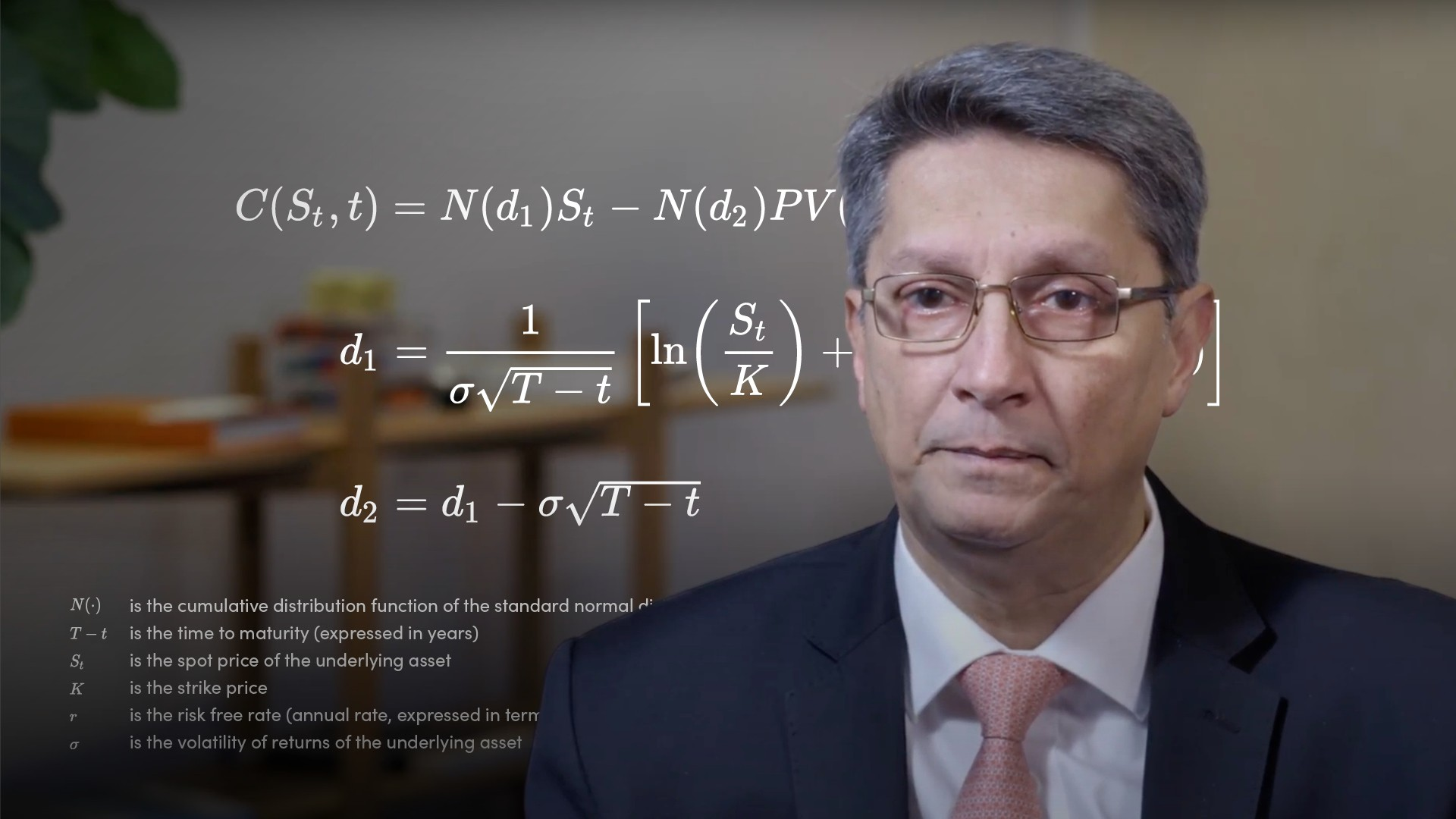05:08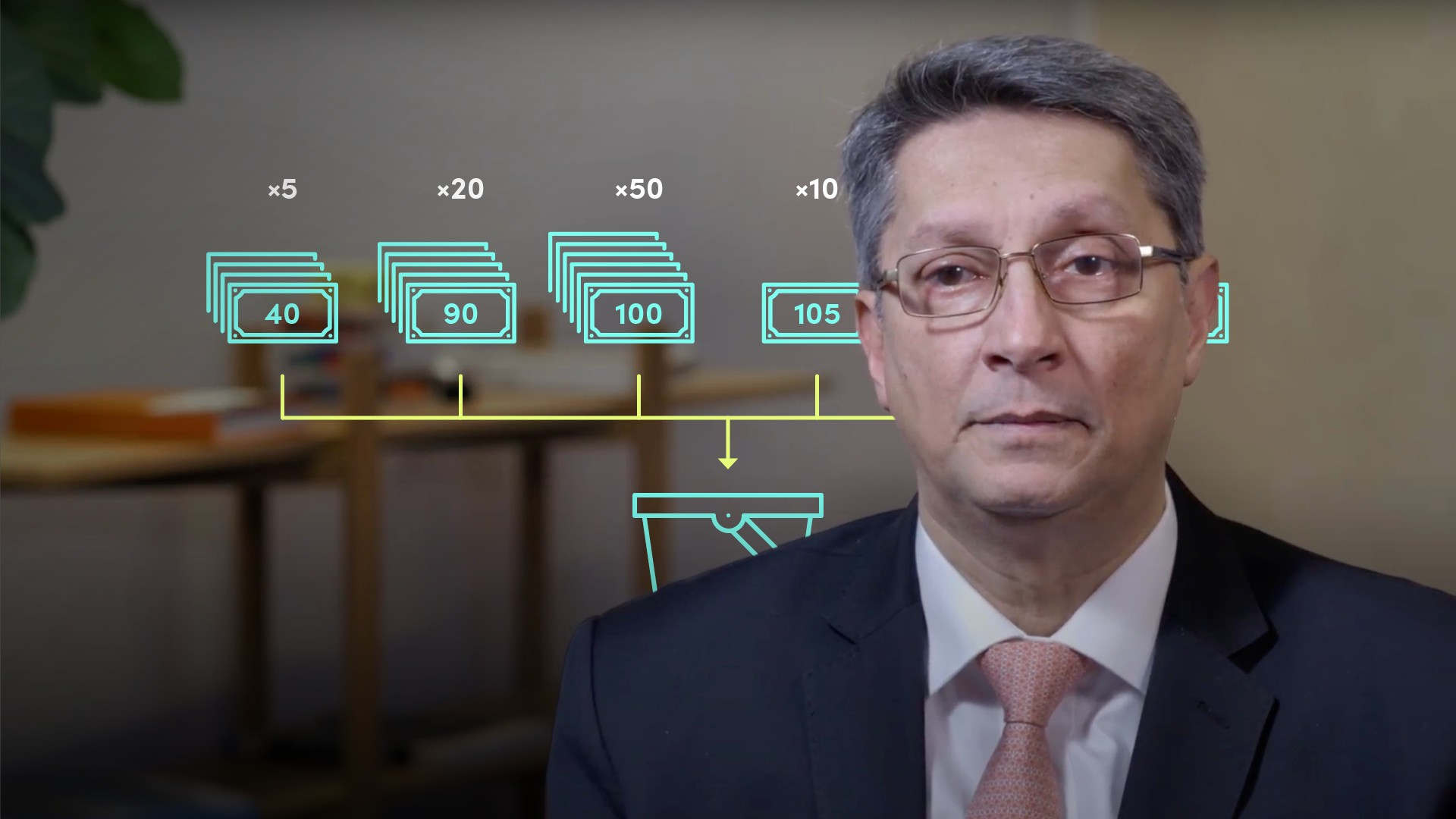04:52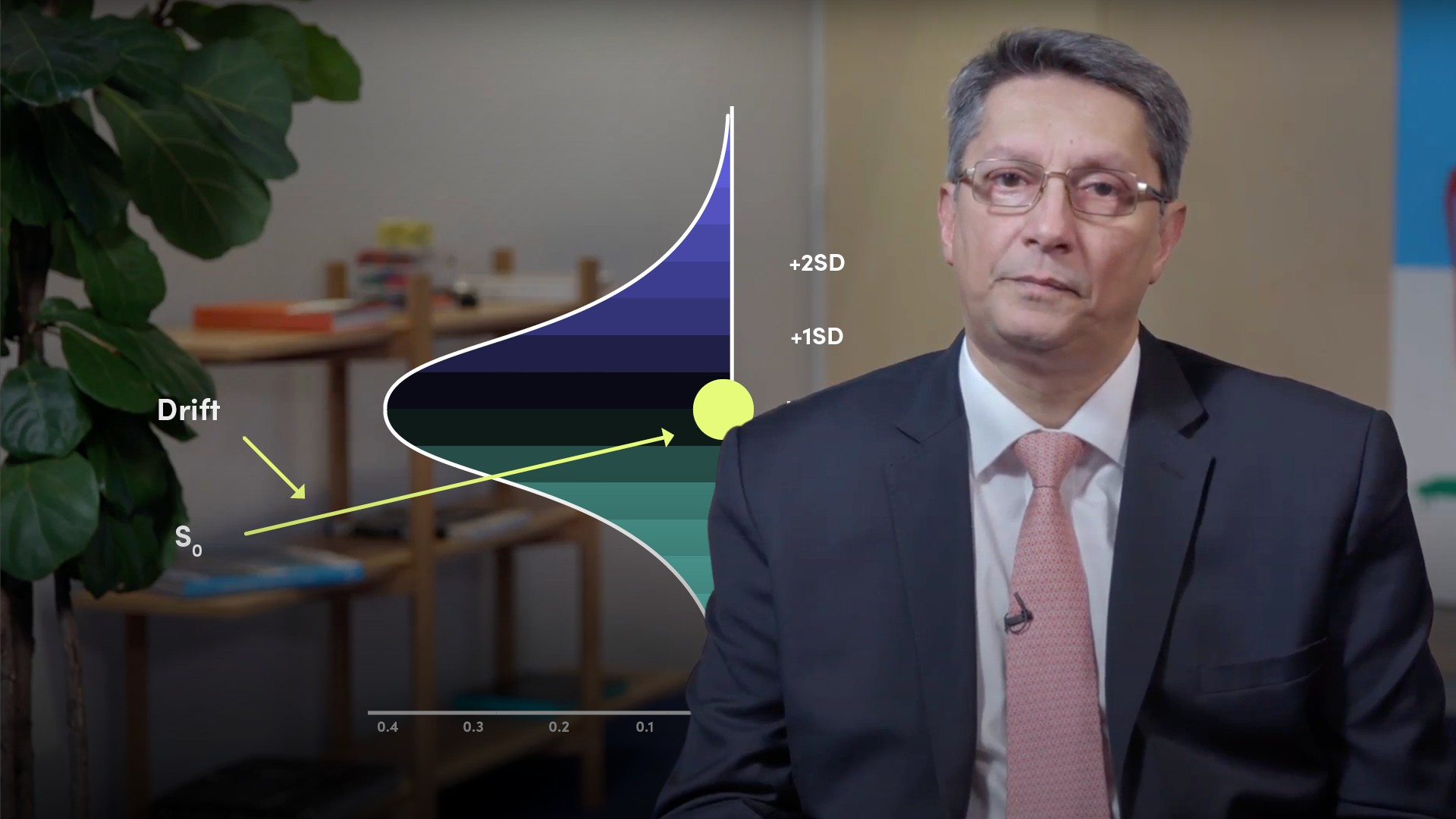05:09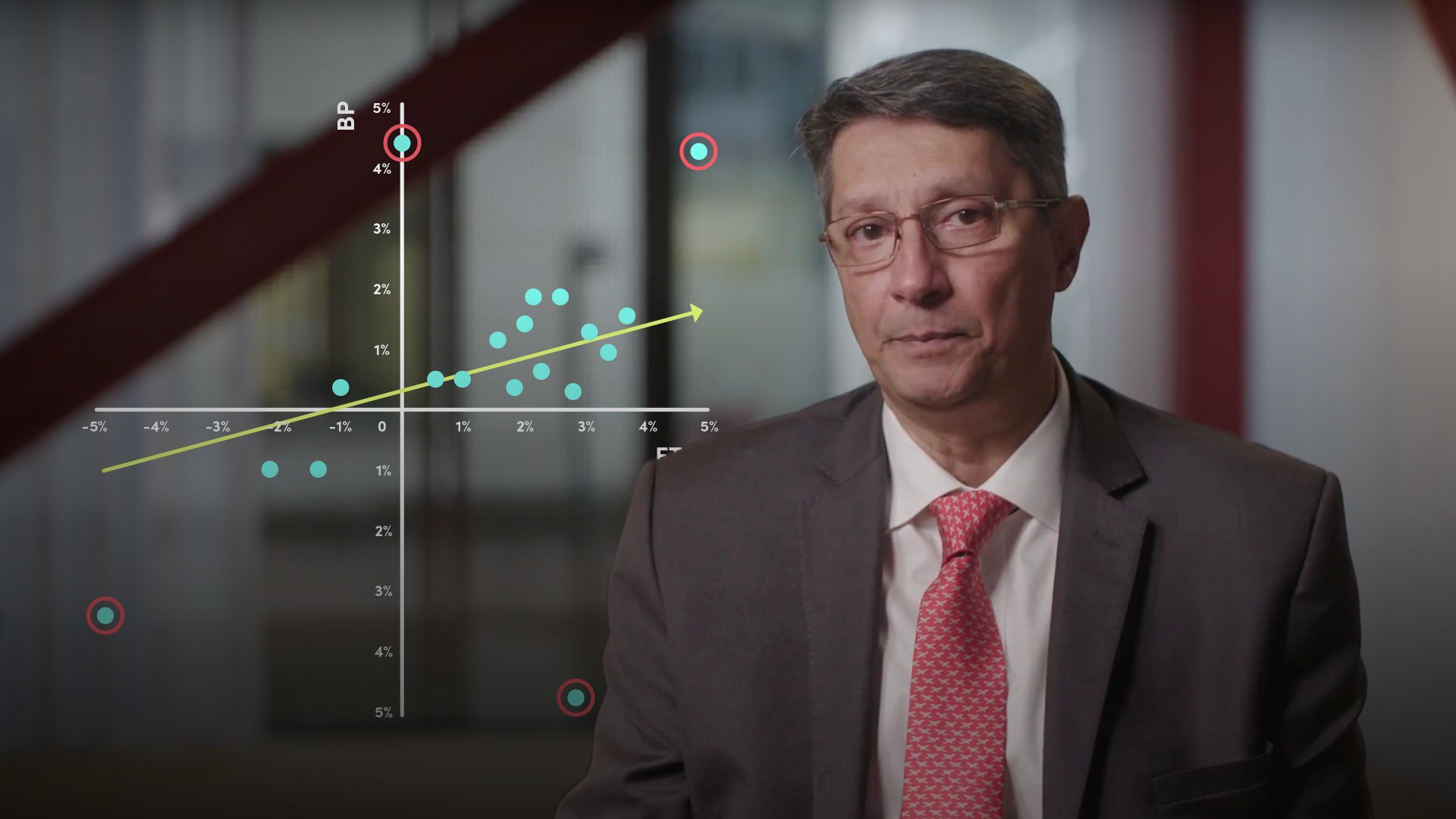04:13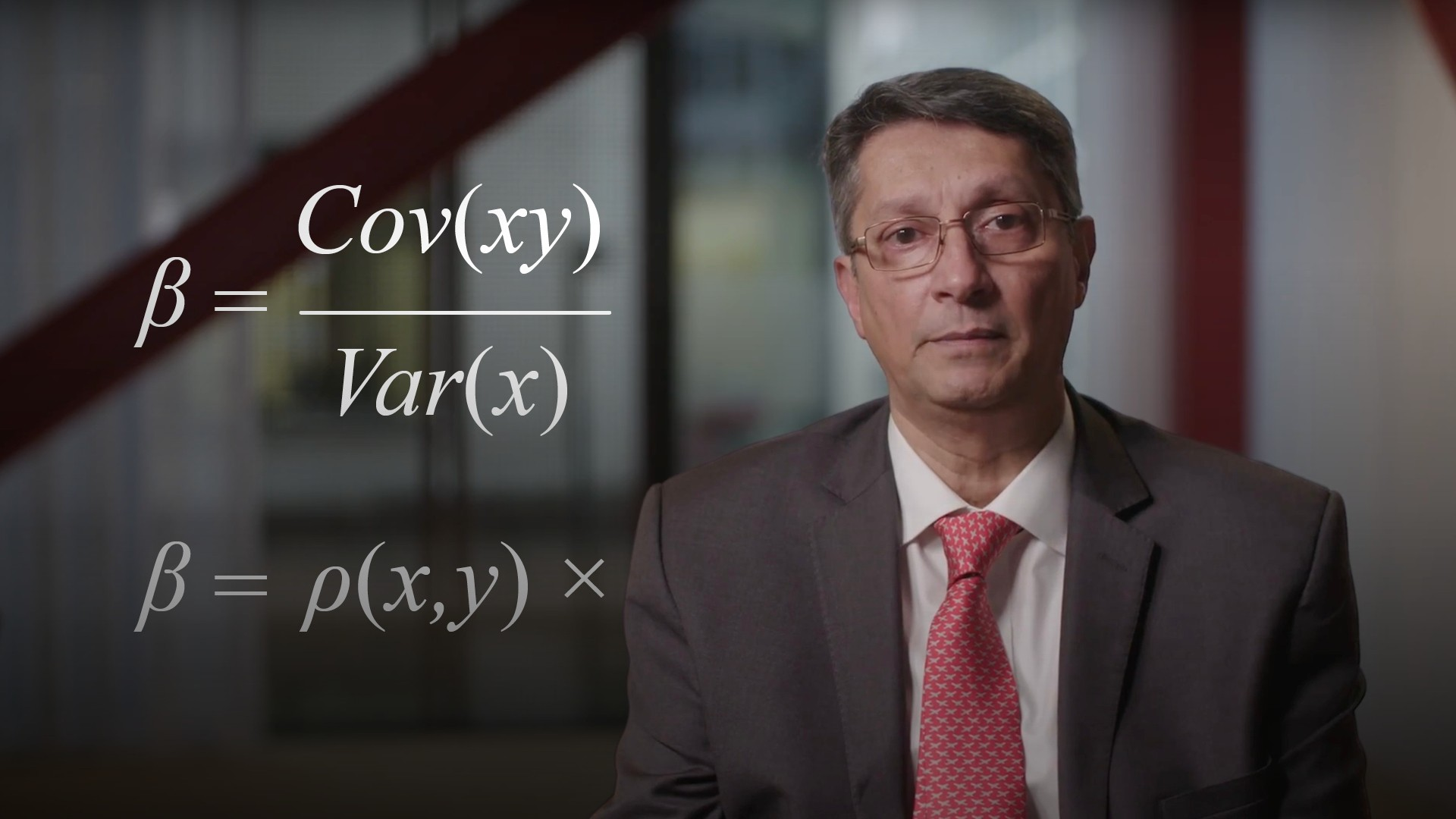05:3804:08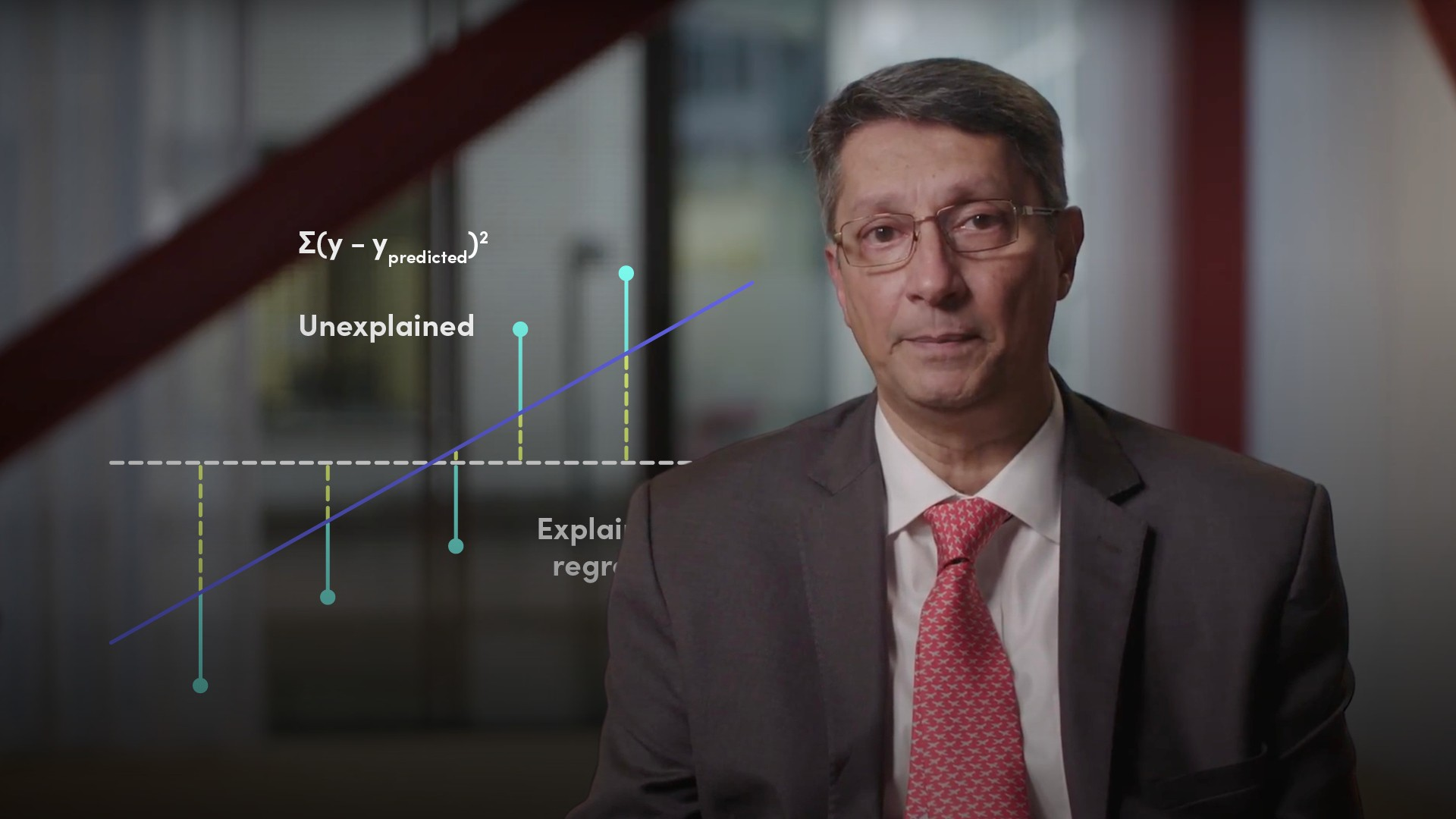04:22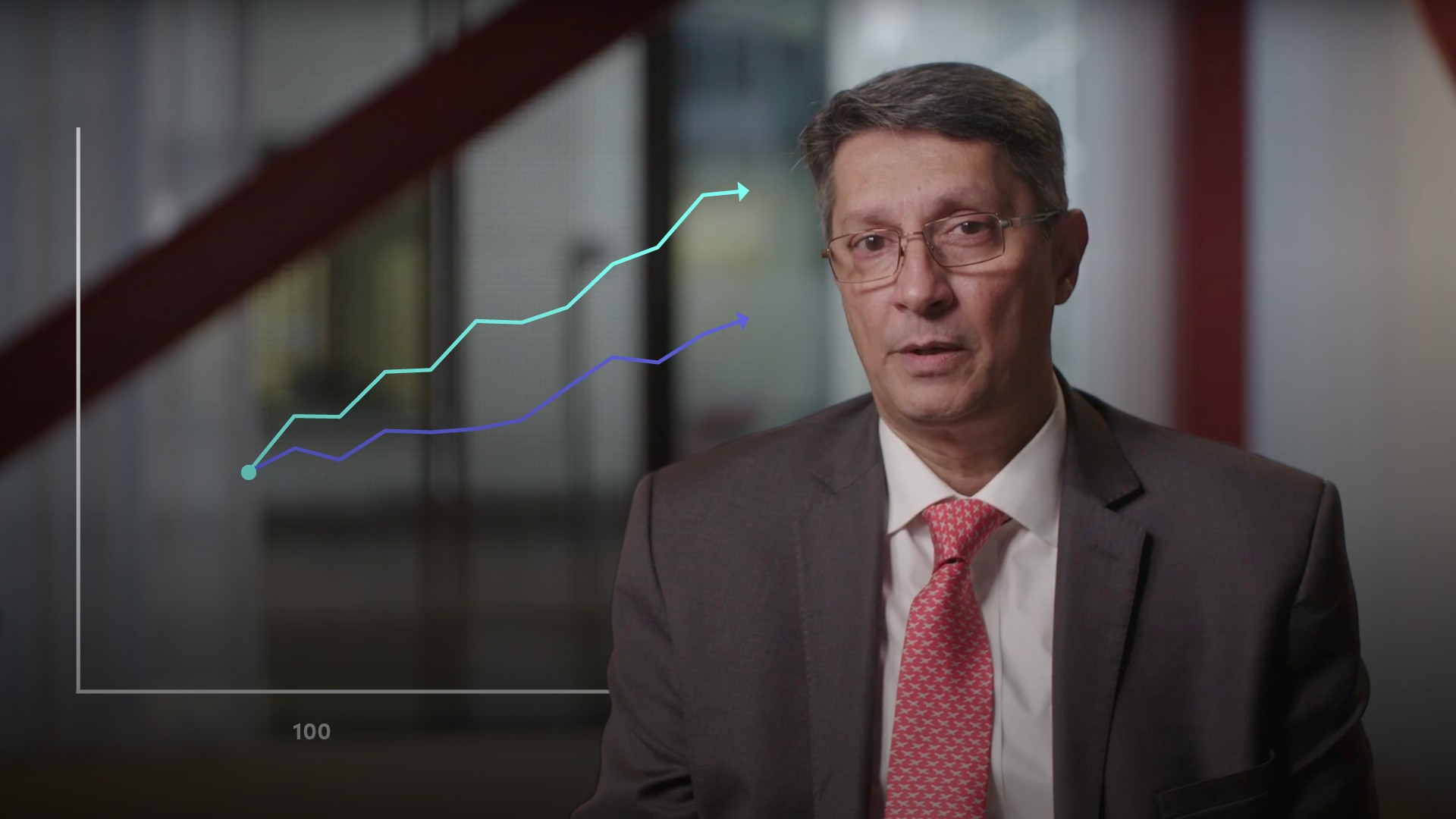05:02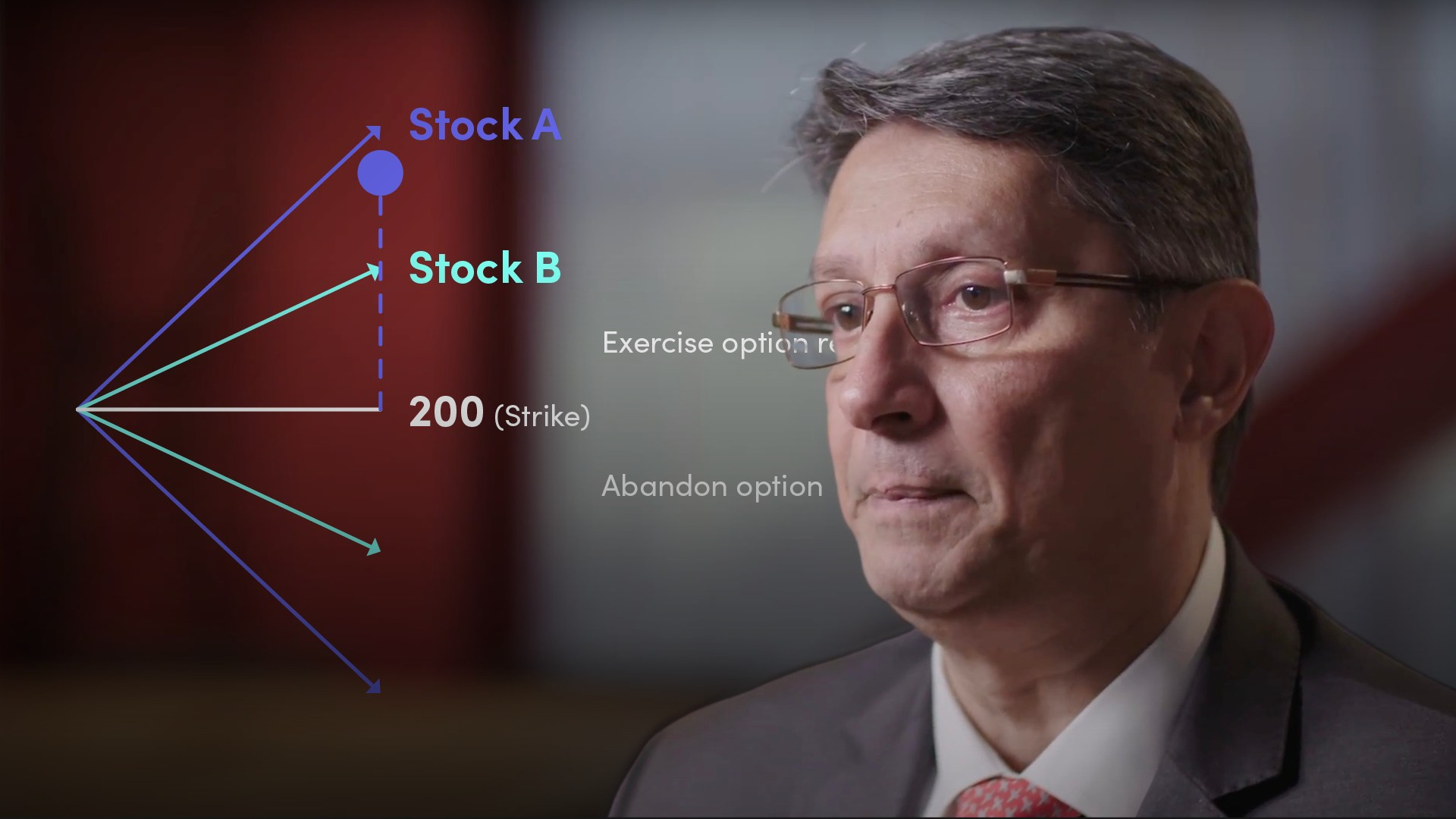03:26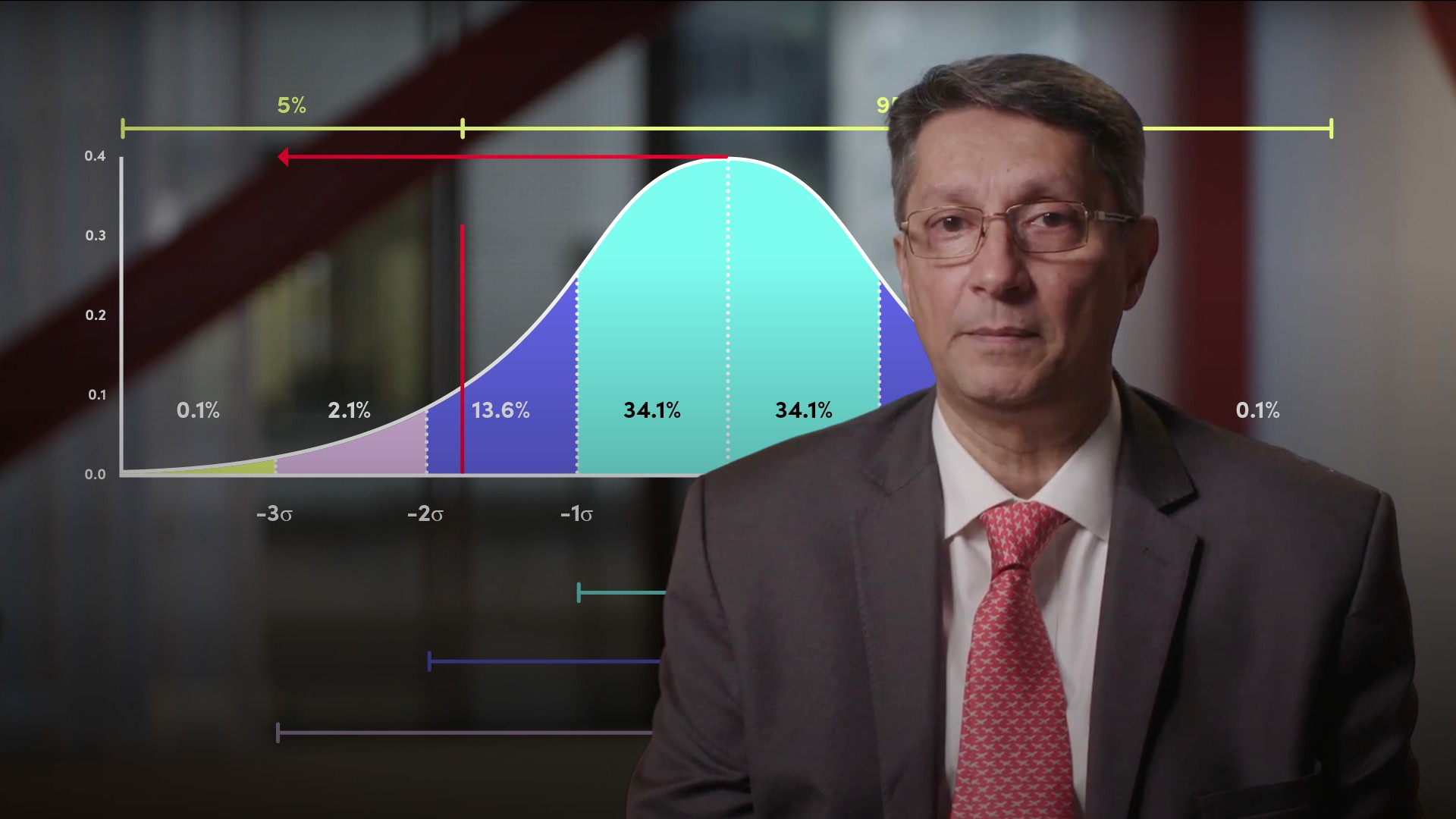03:58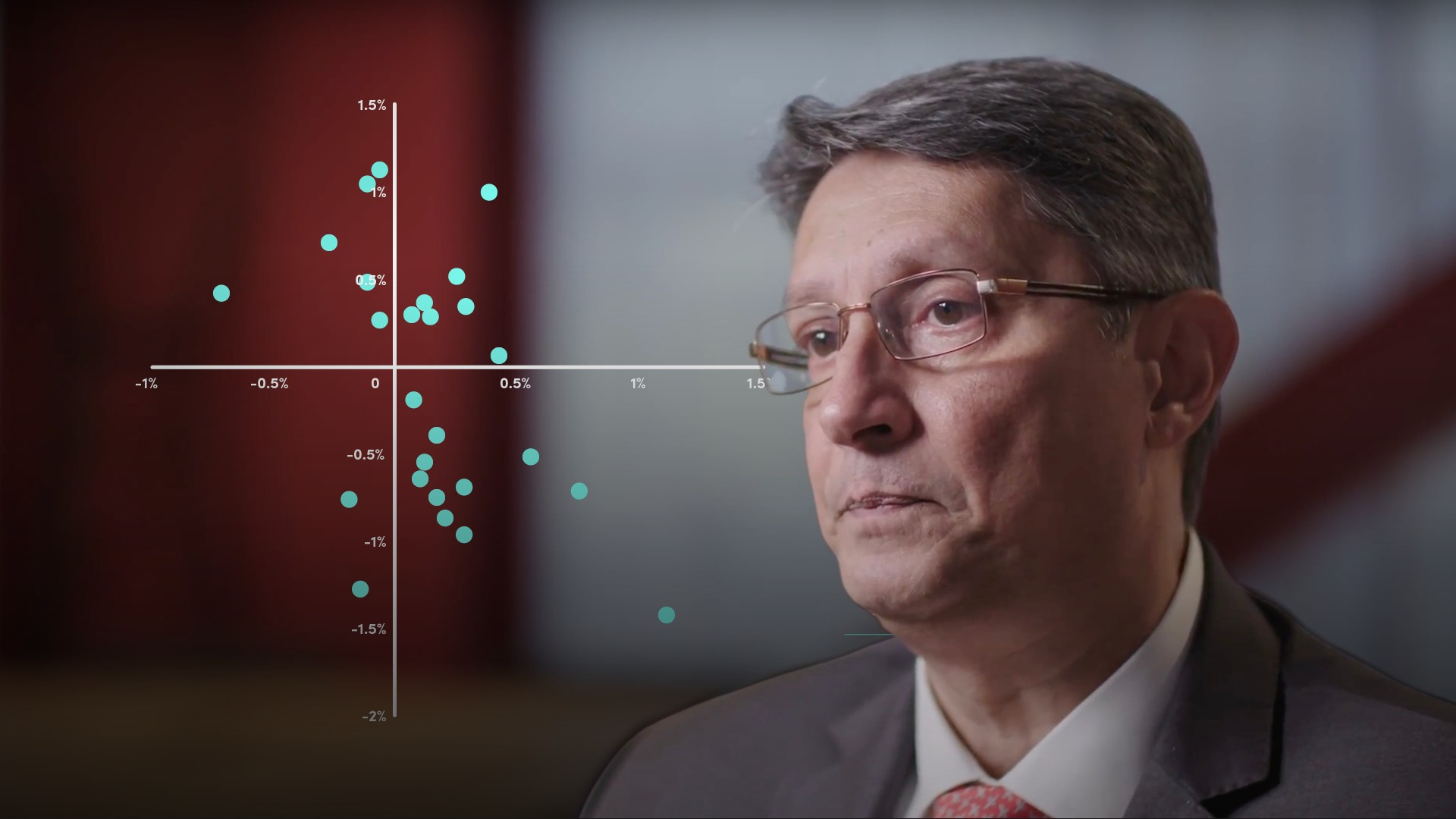04:55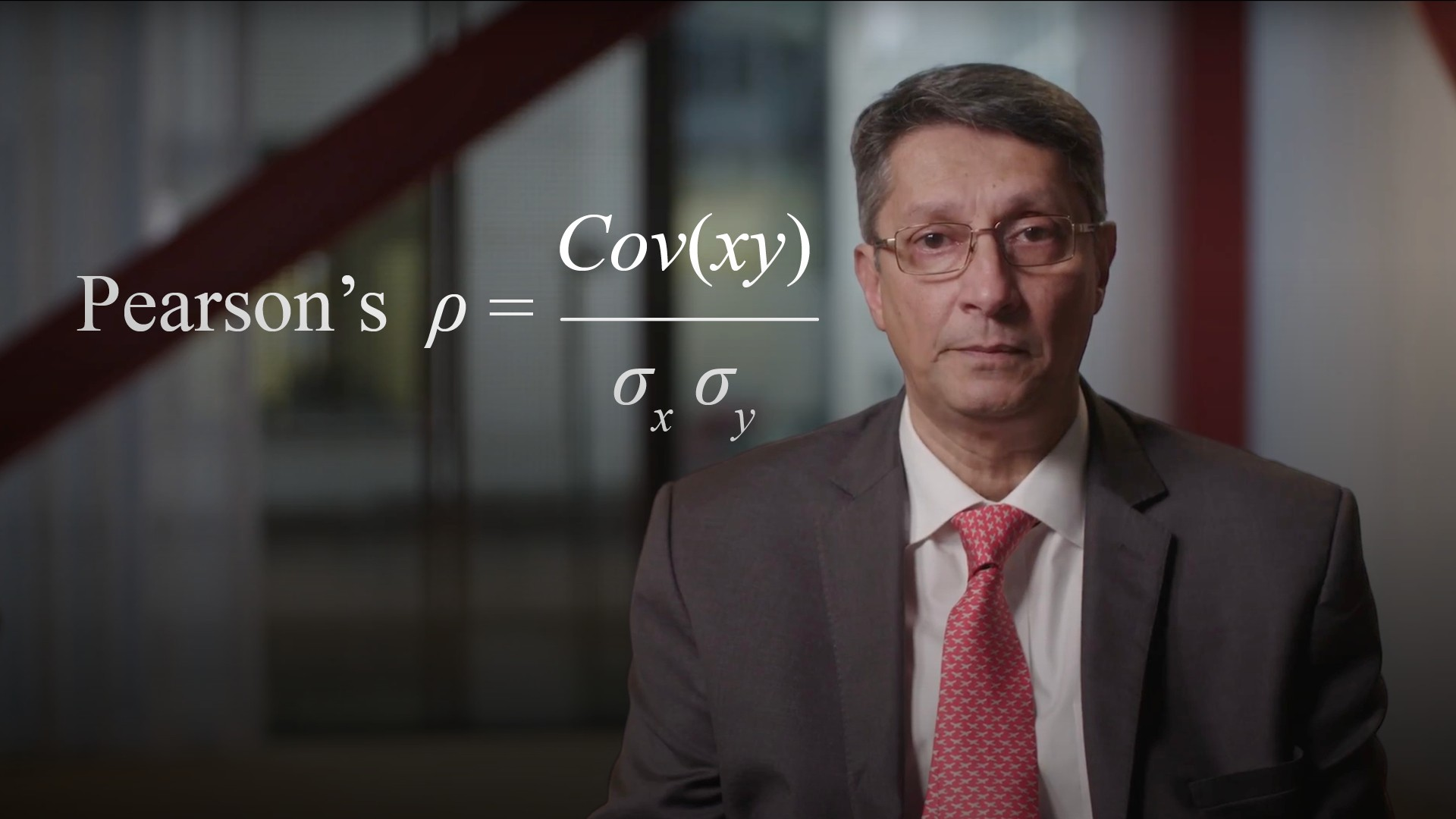04:56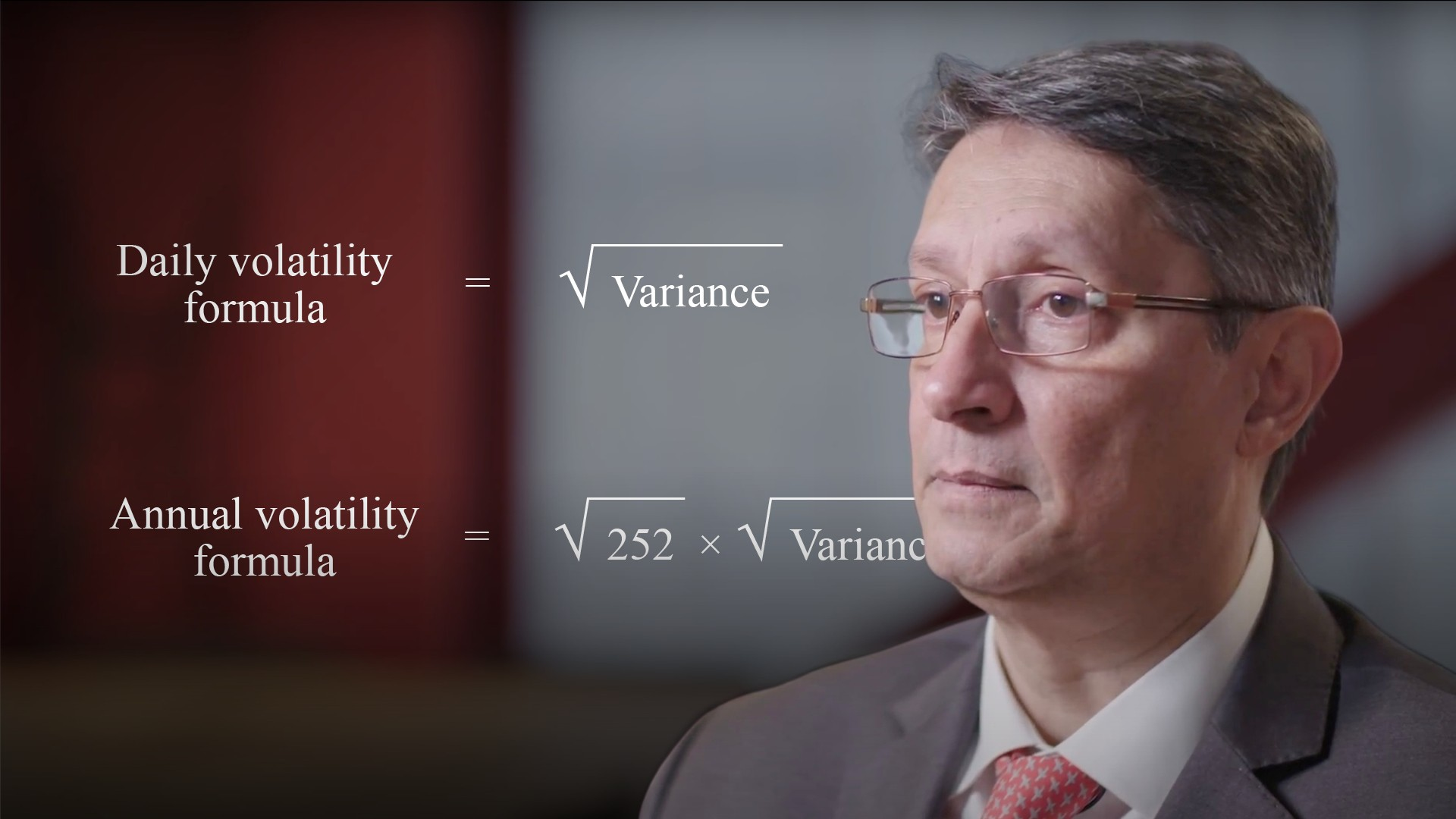04:34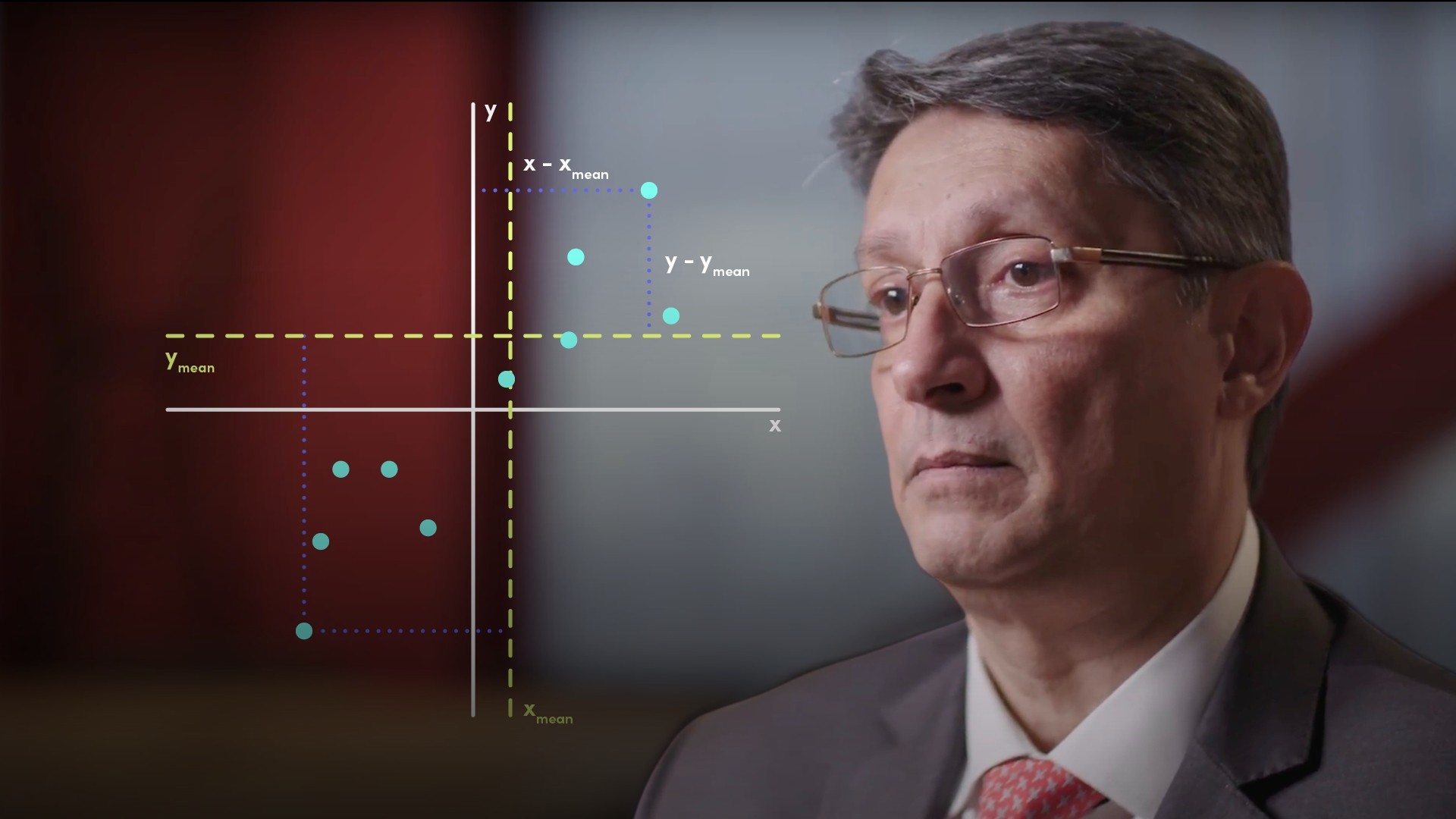04:40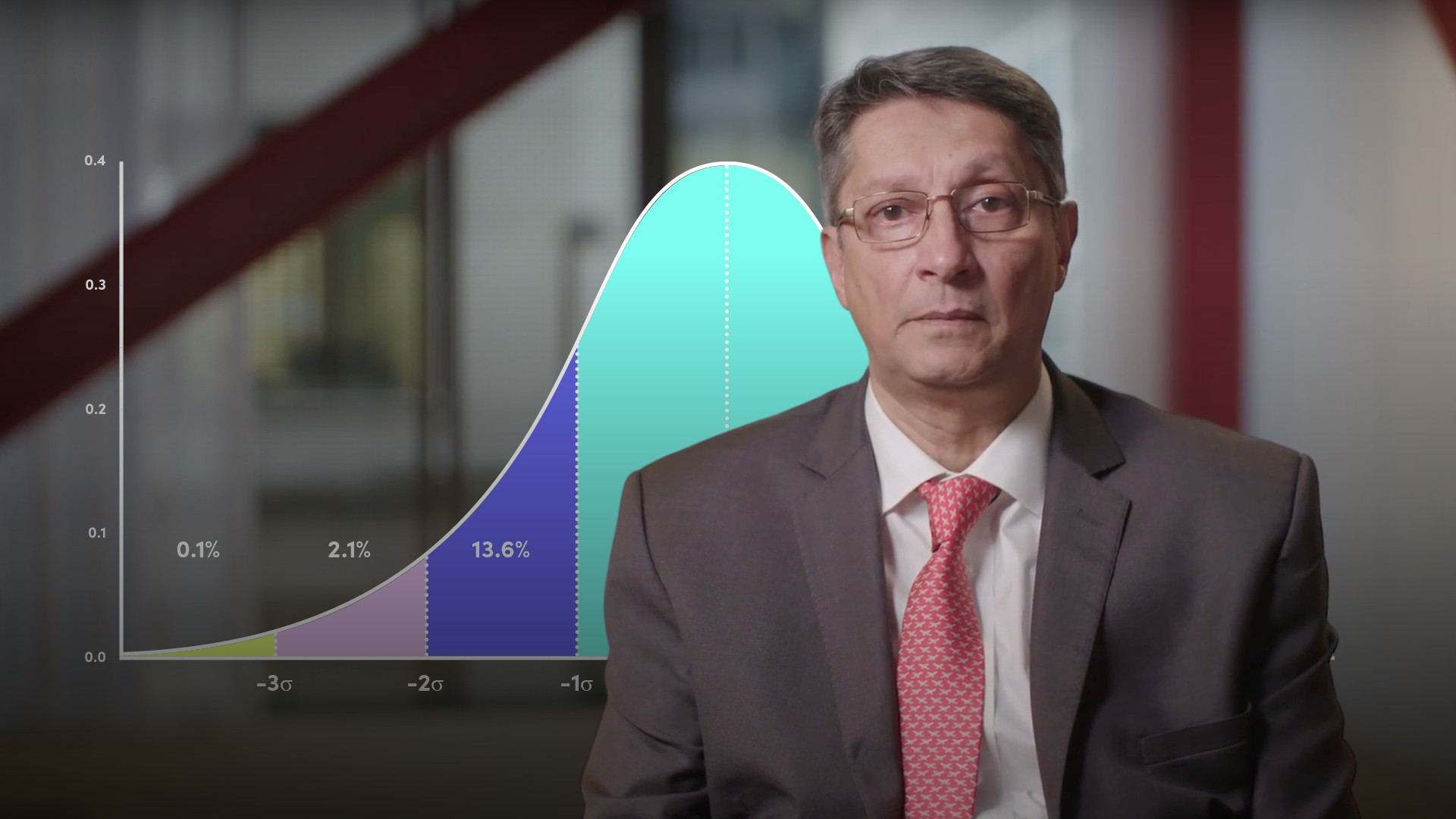05:42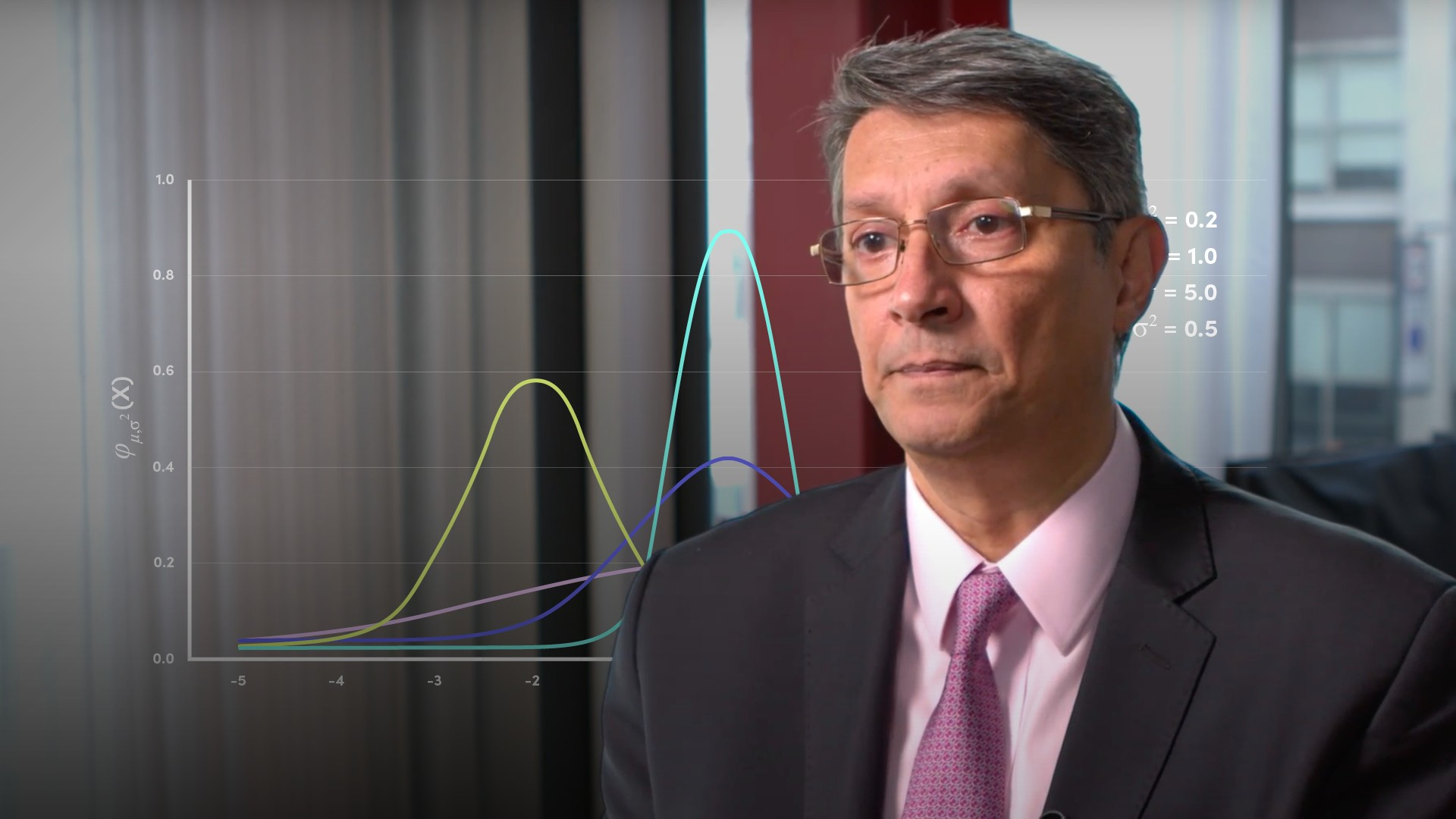04:49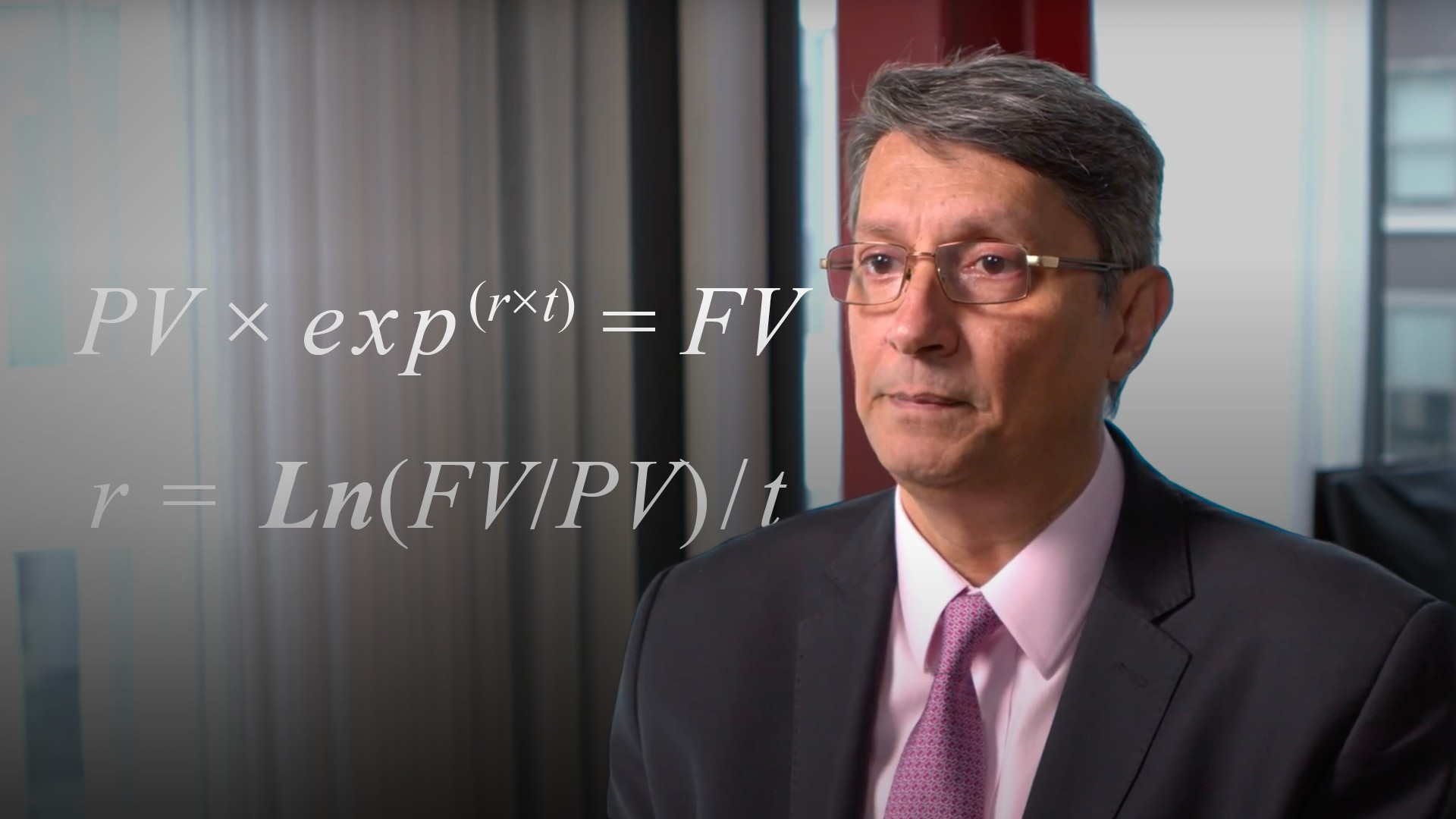03:5806:44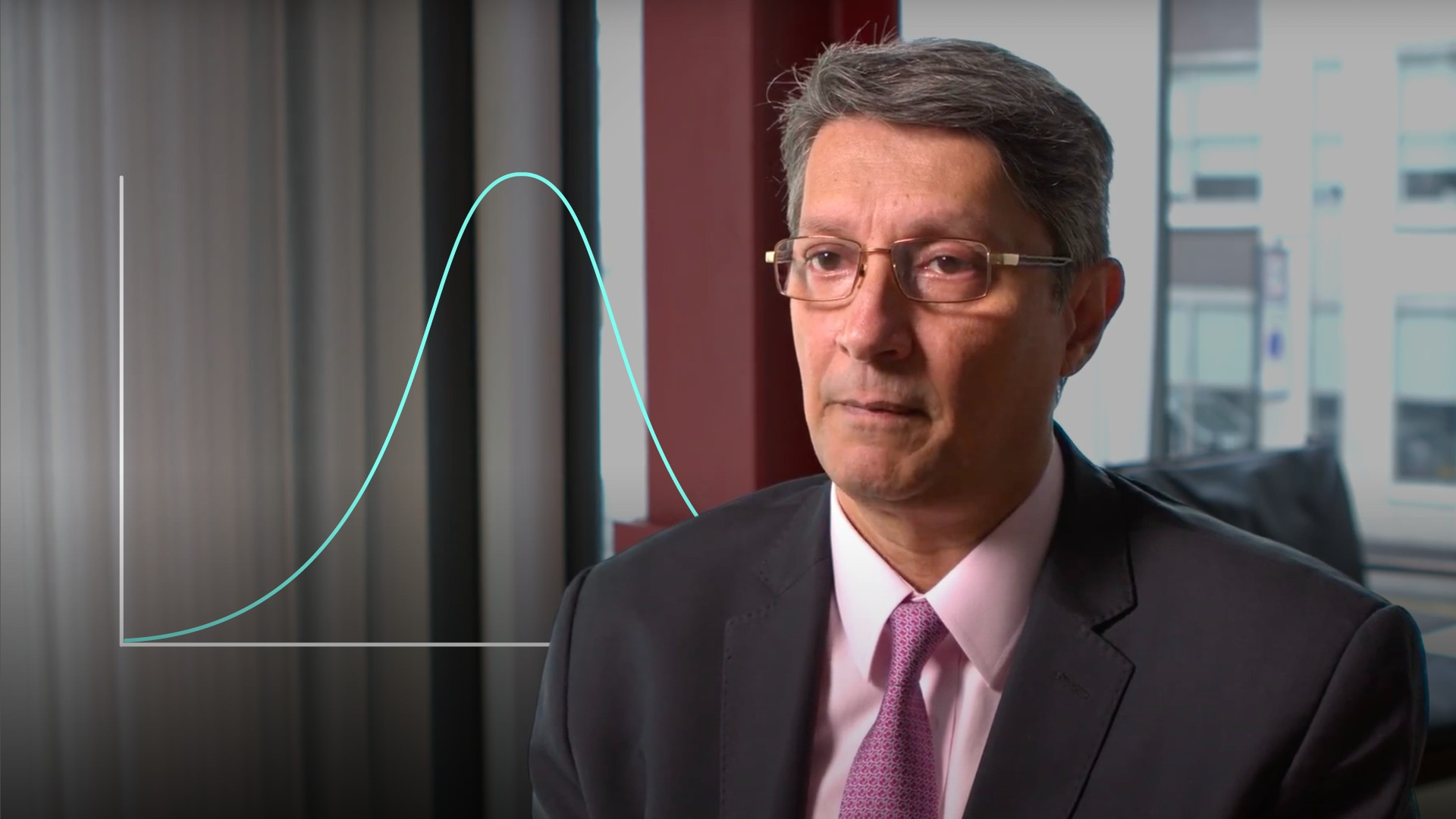03:49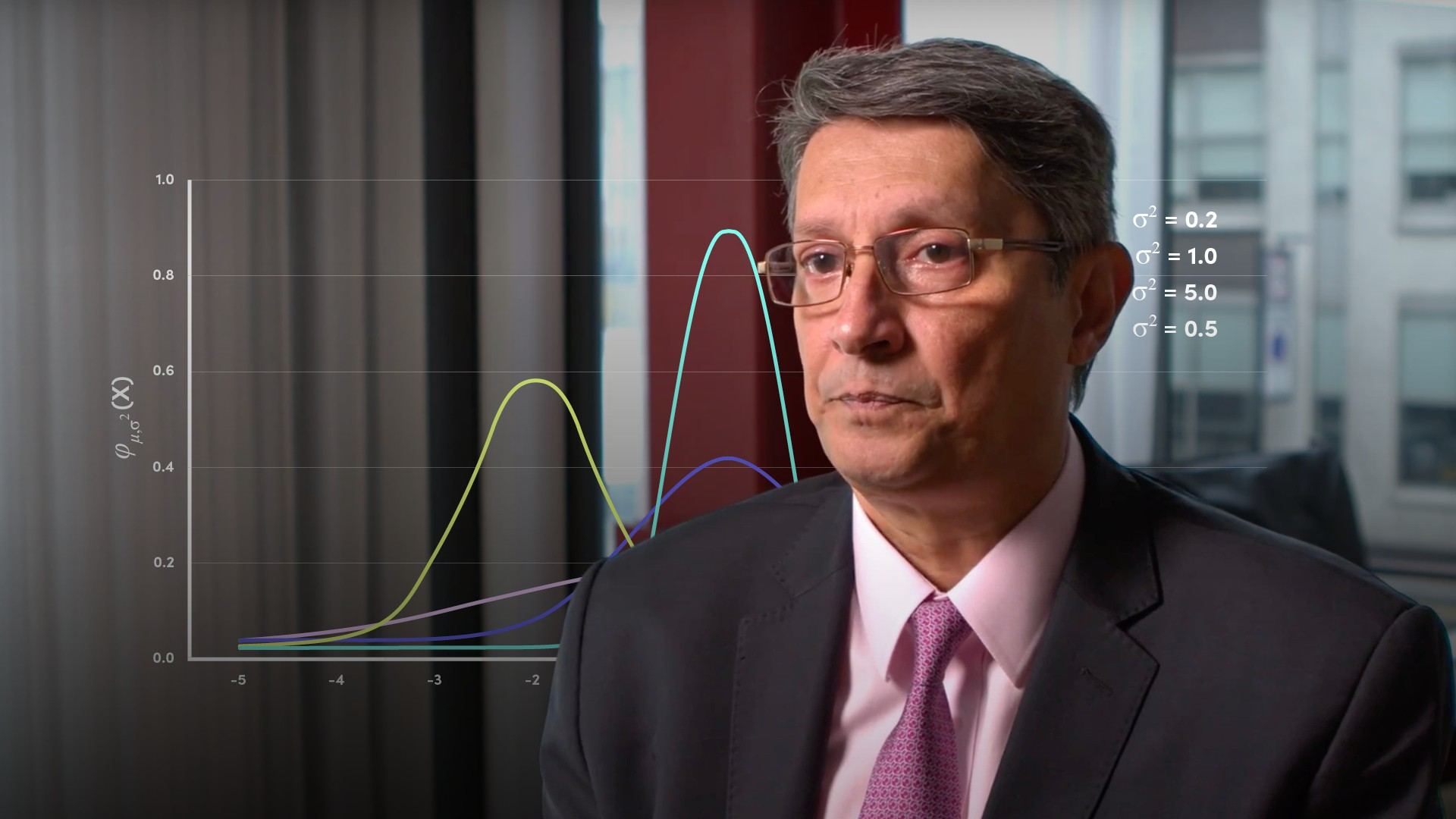04:57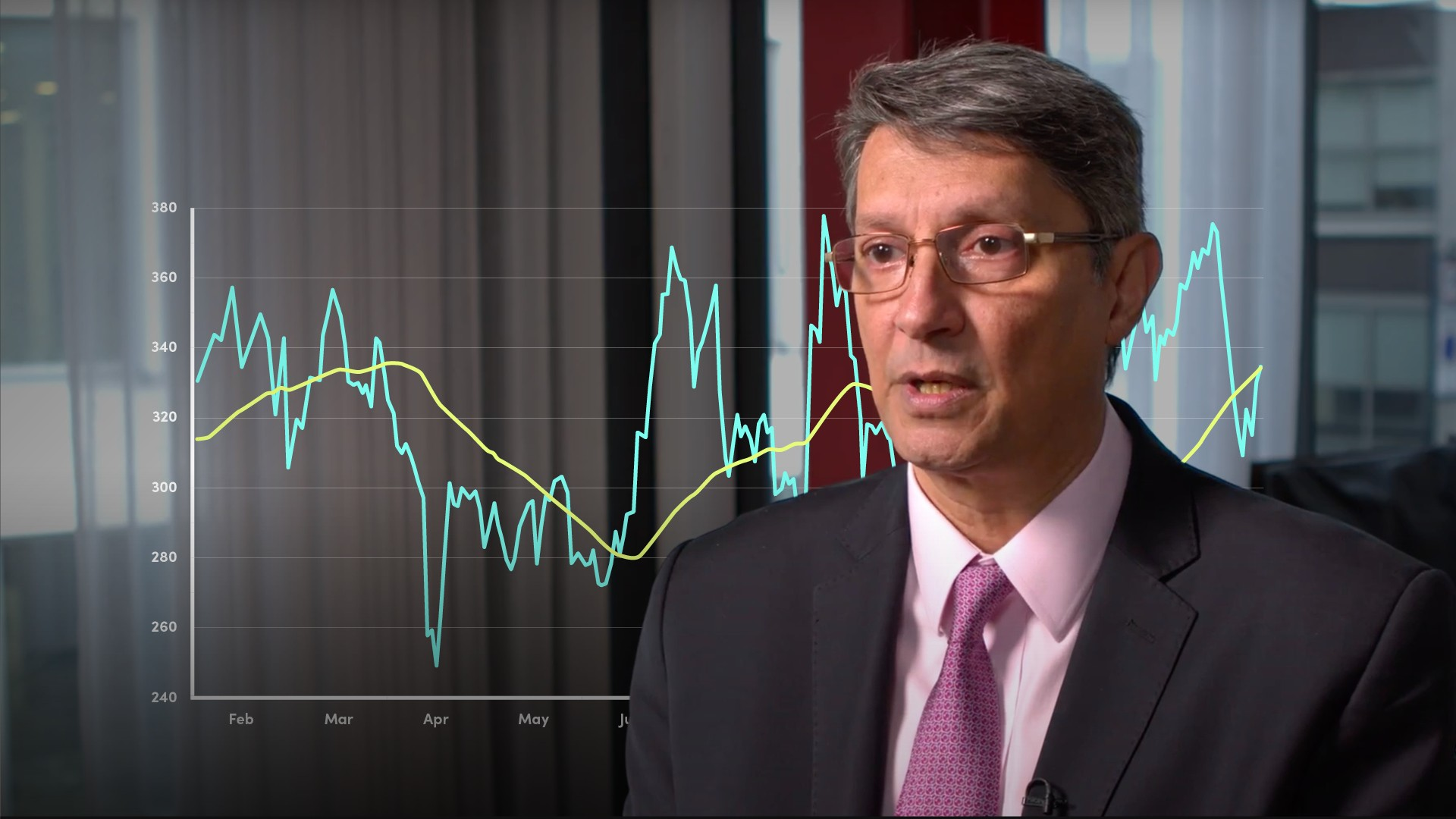04:0004:11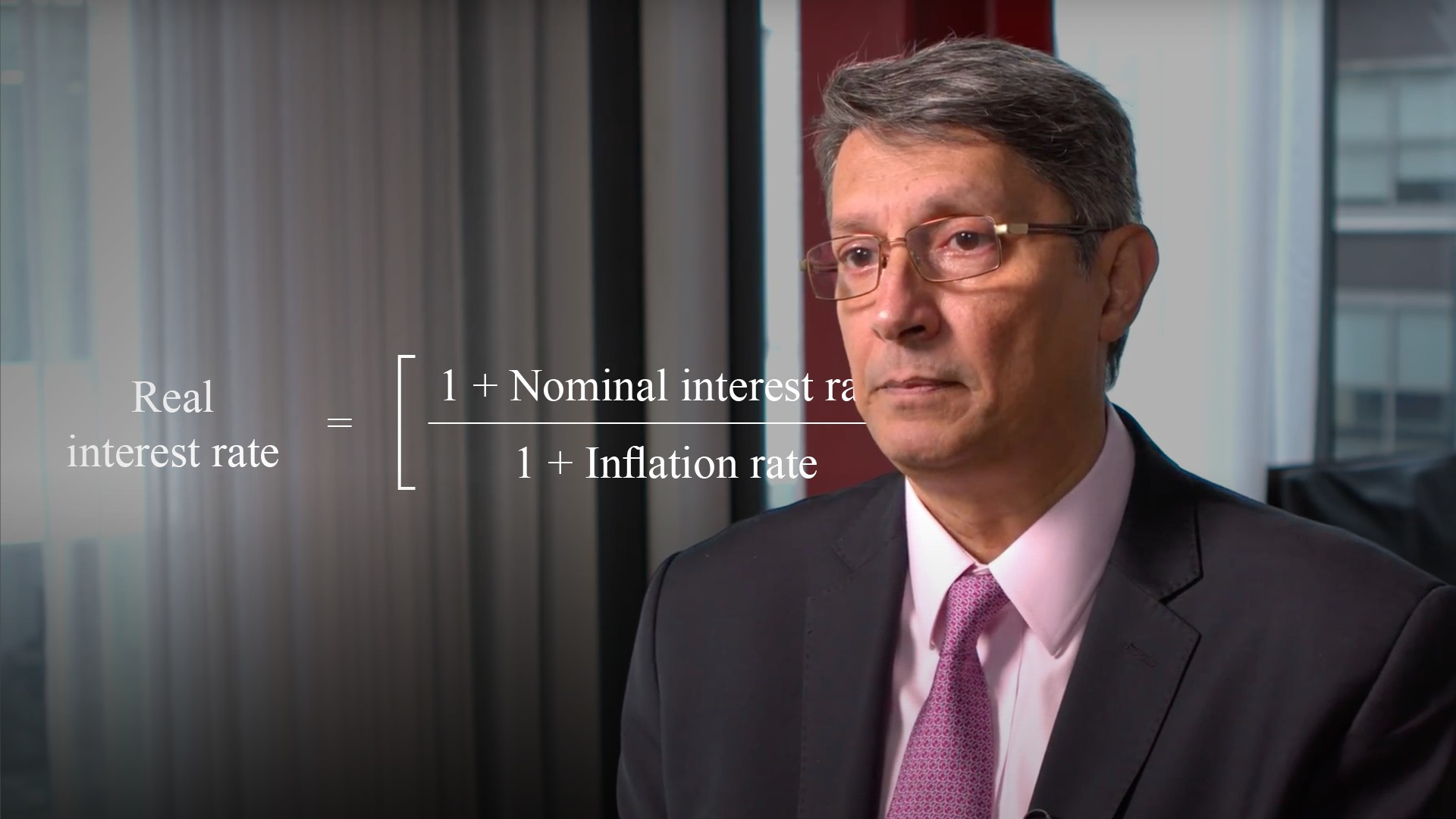04:42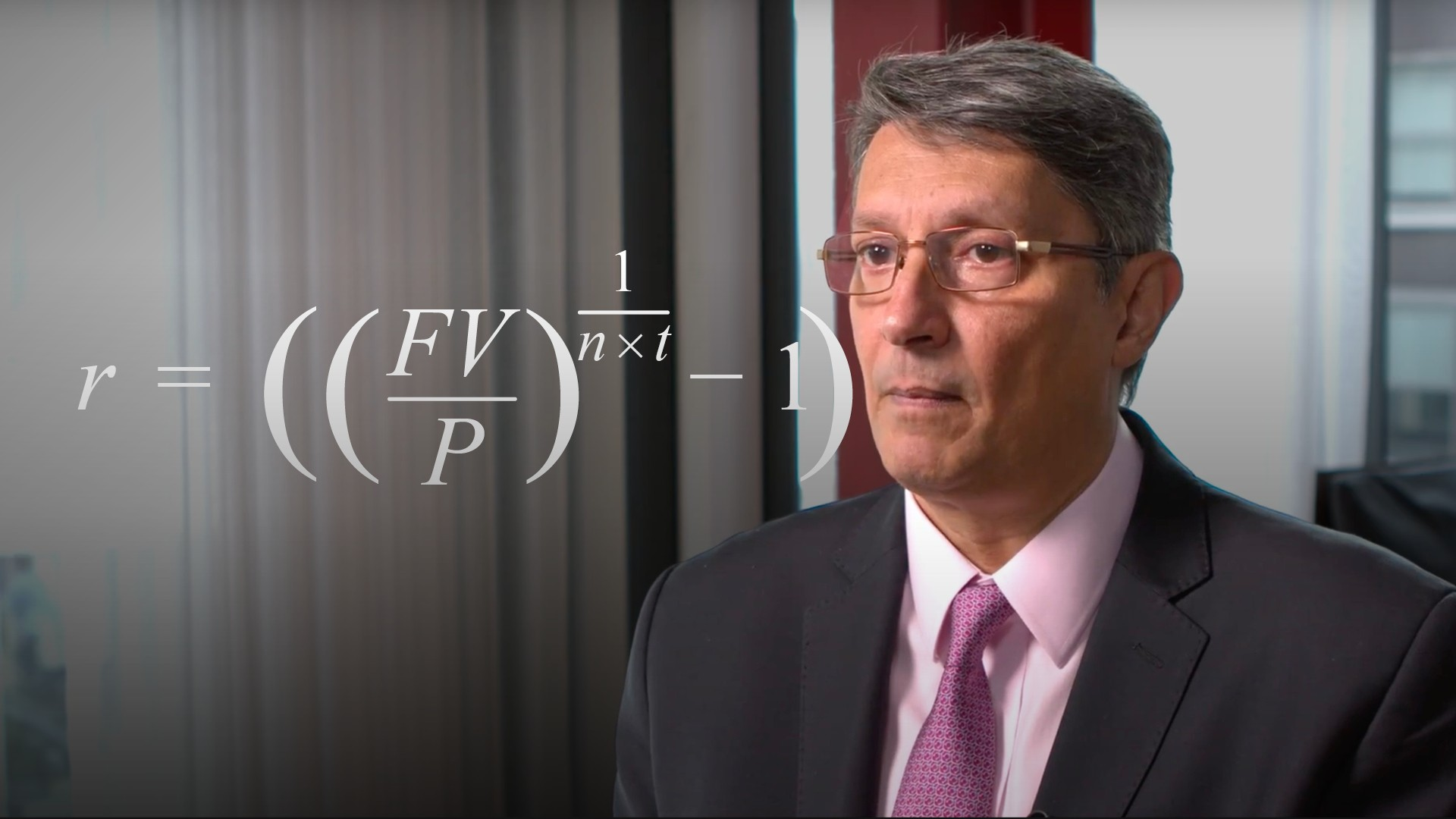05:03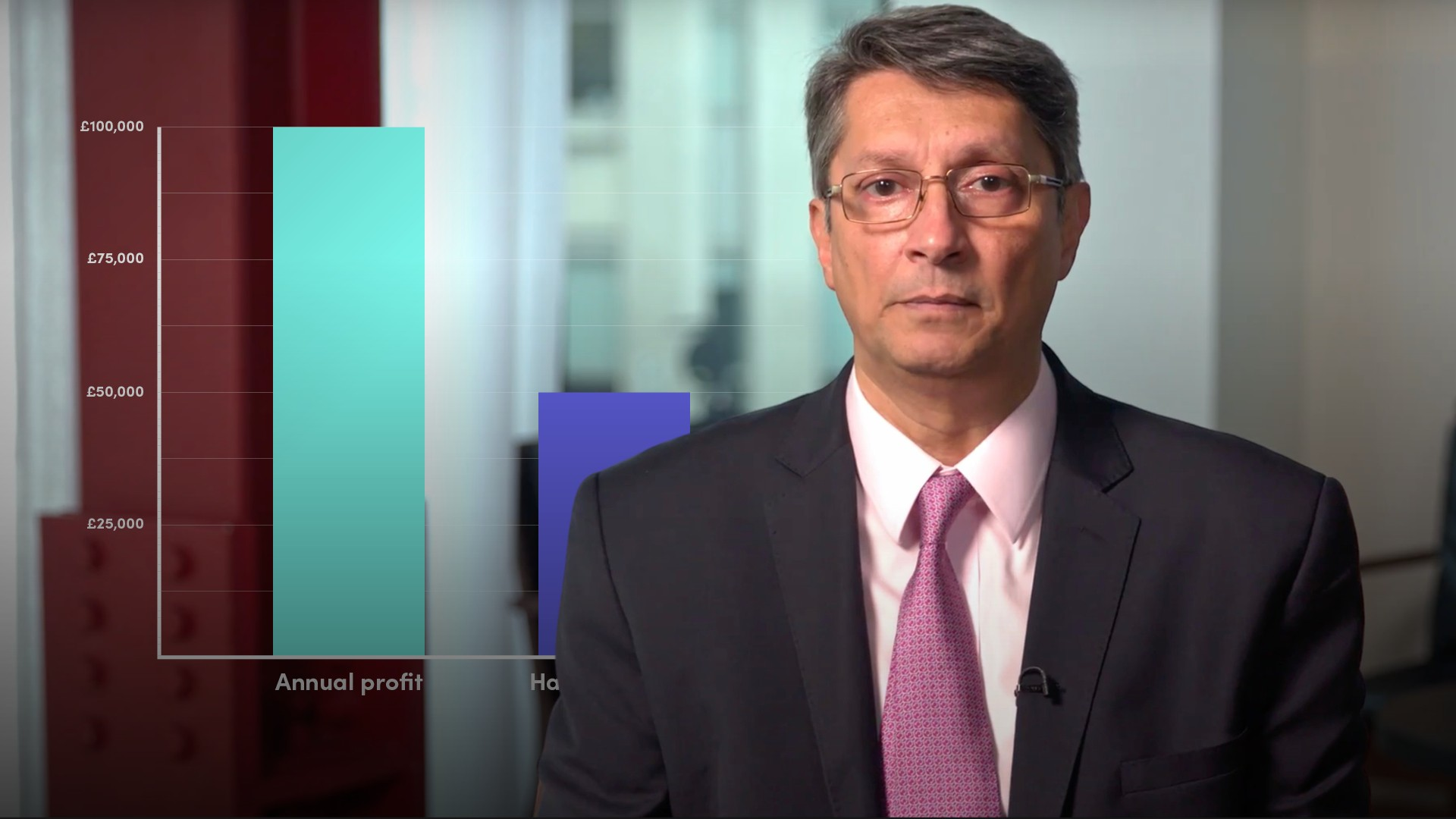04:37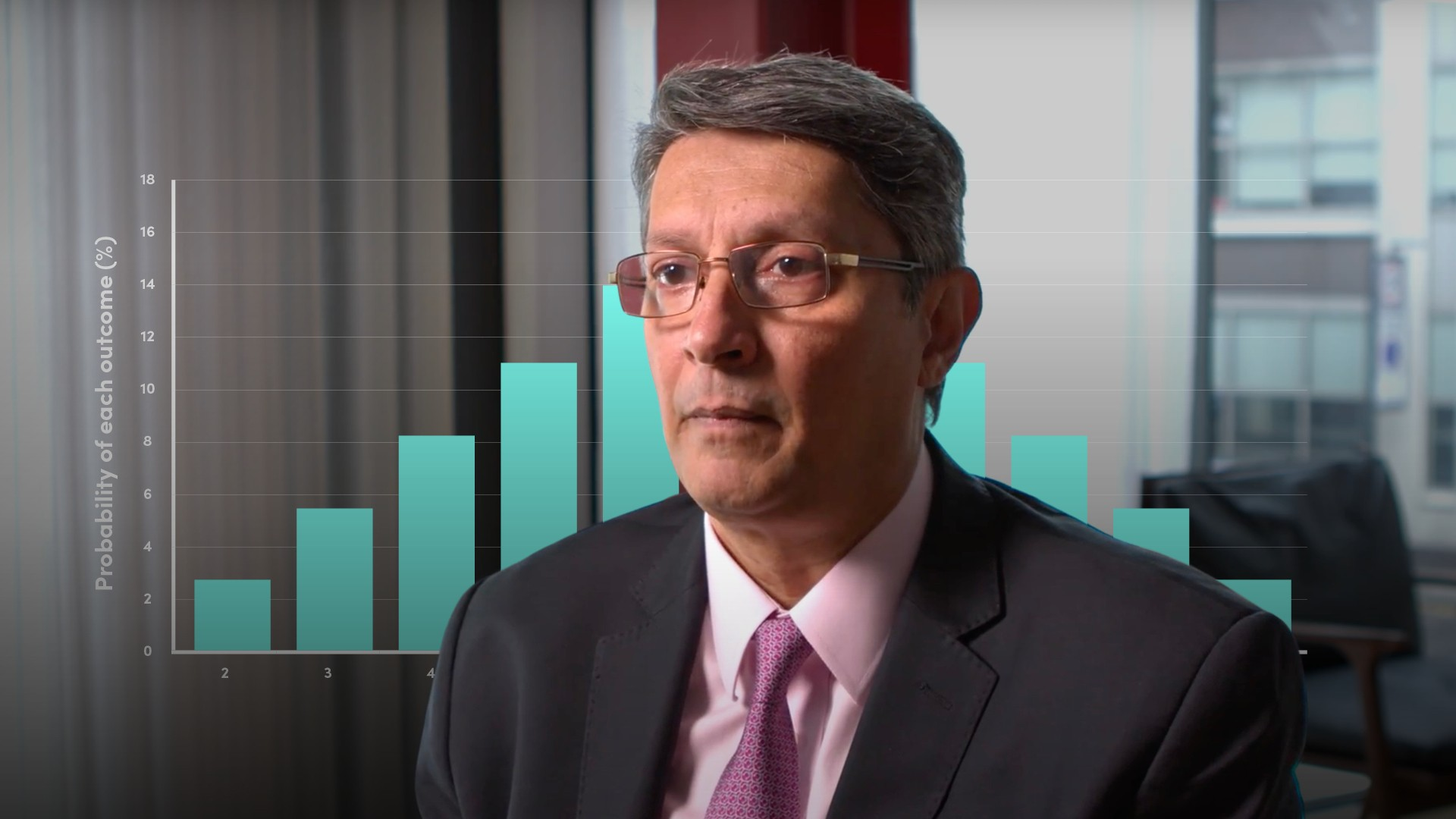04:36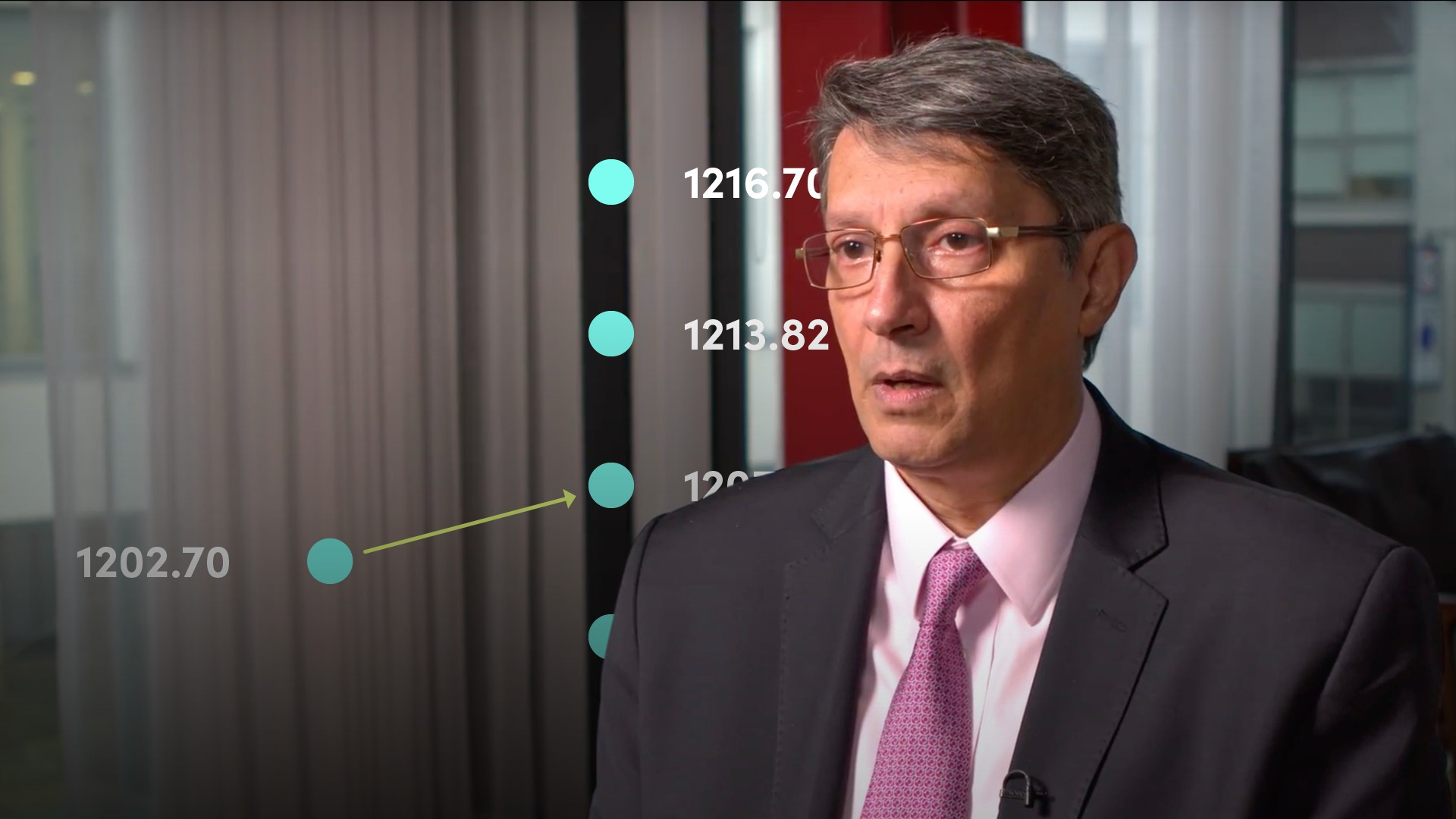06:35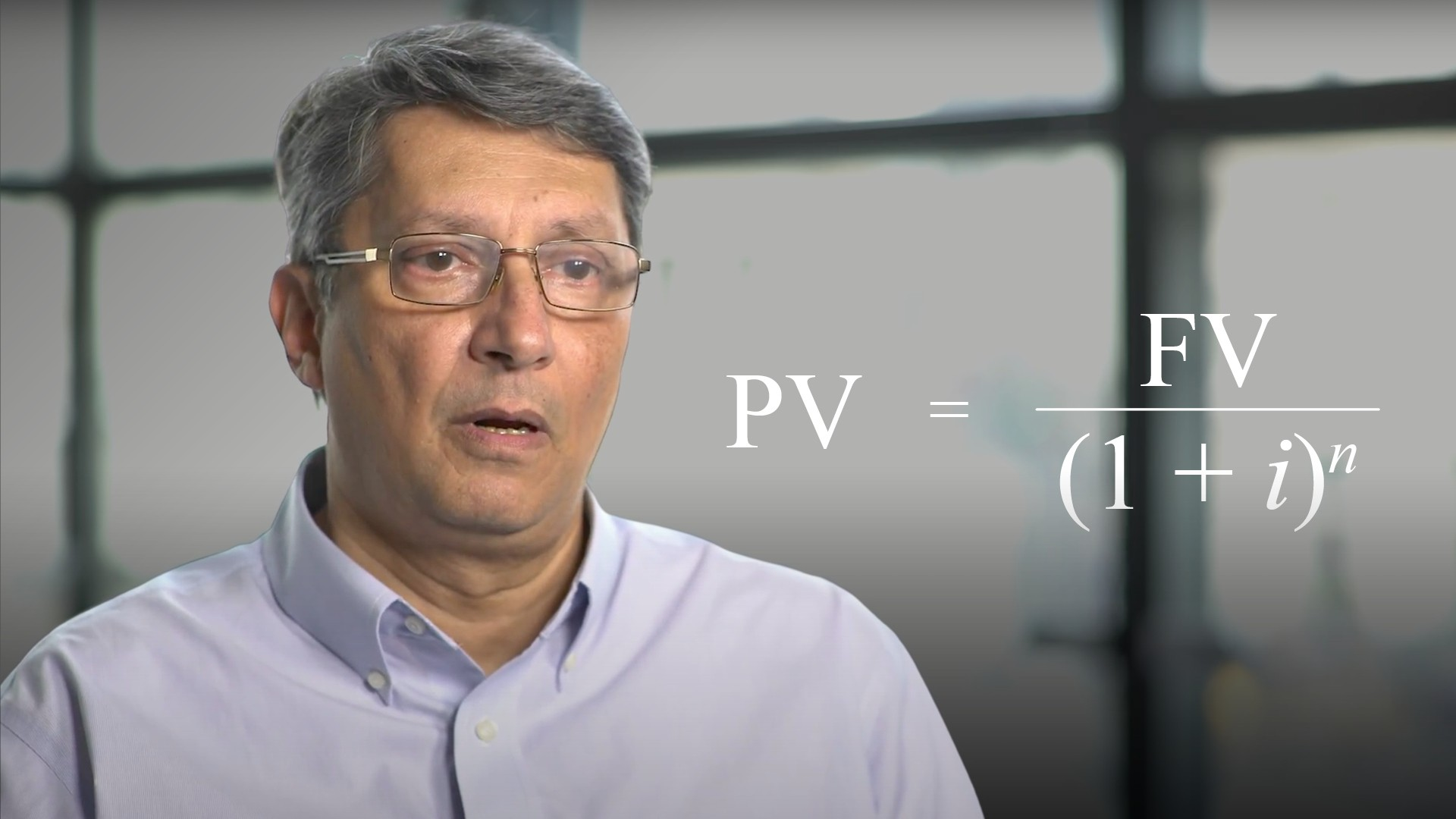05:00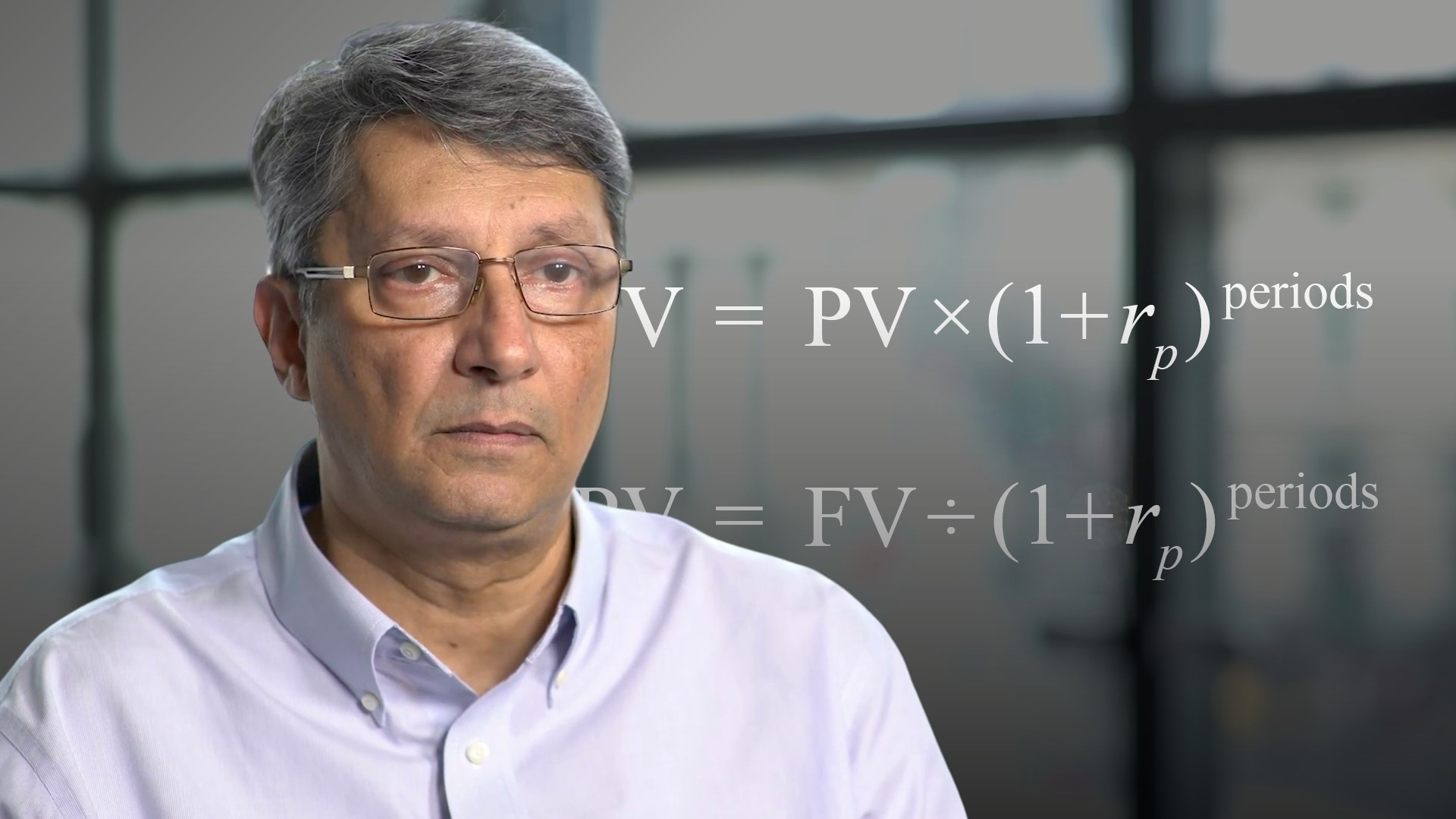03:10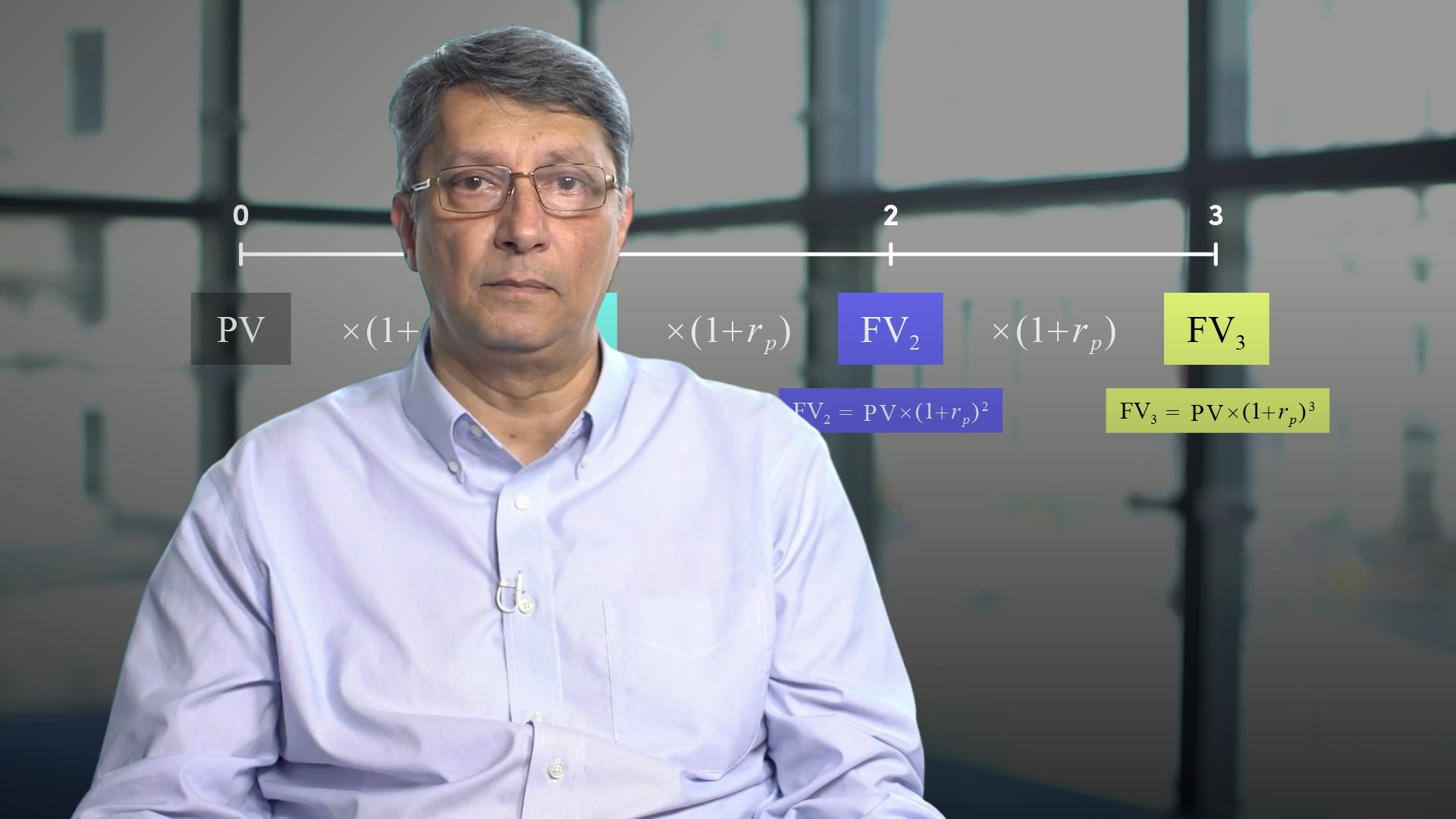06:0805:28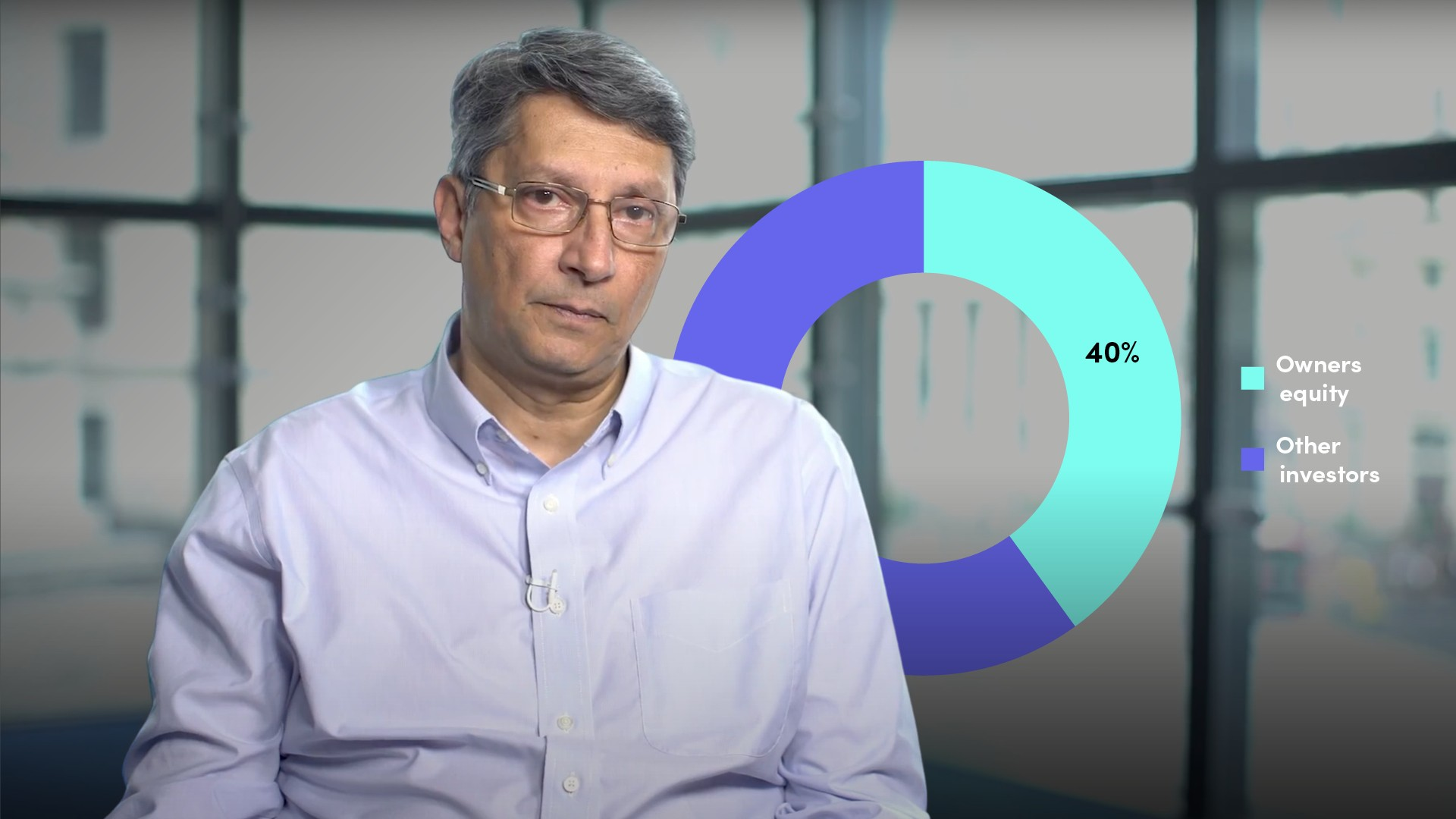05:23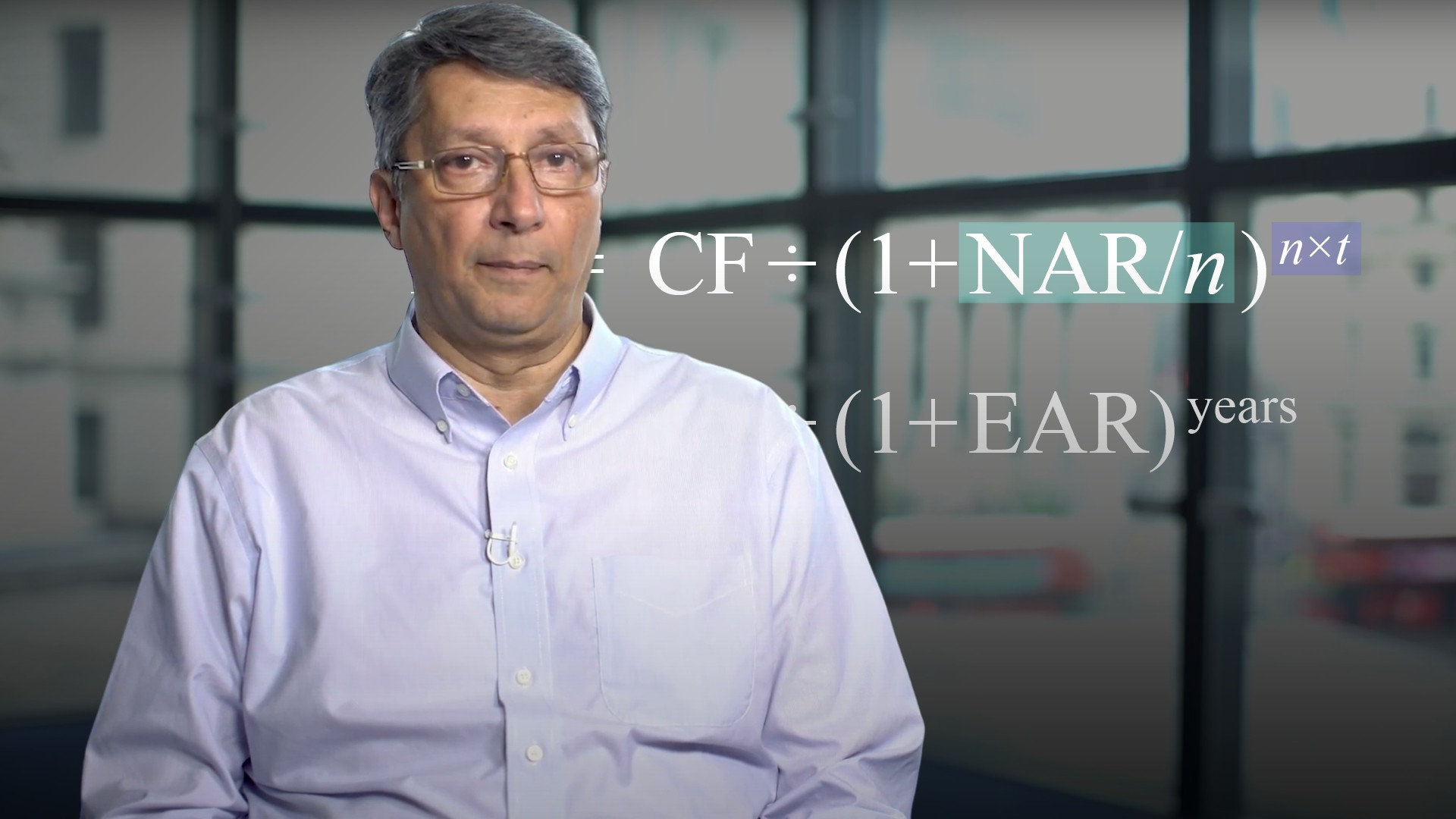04:04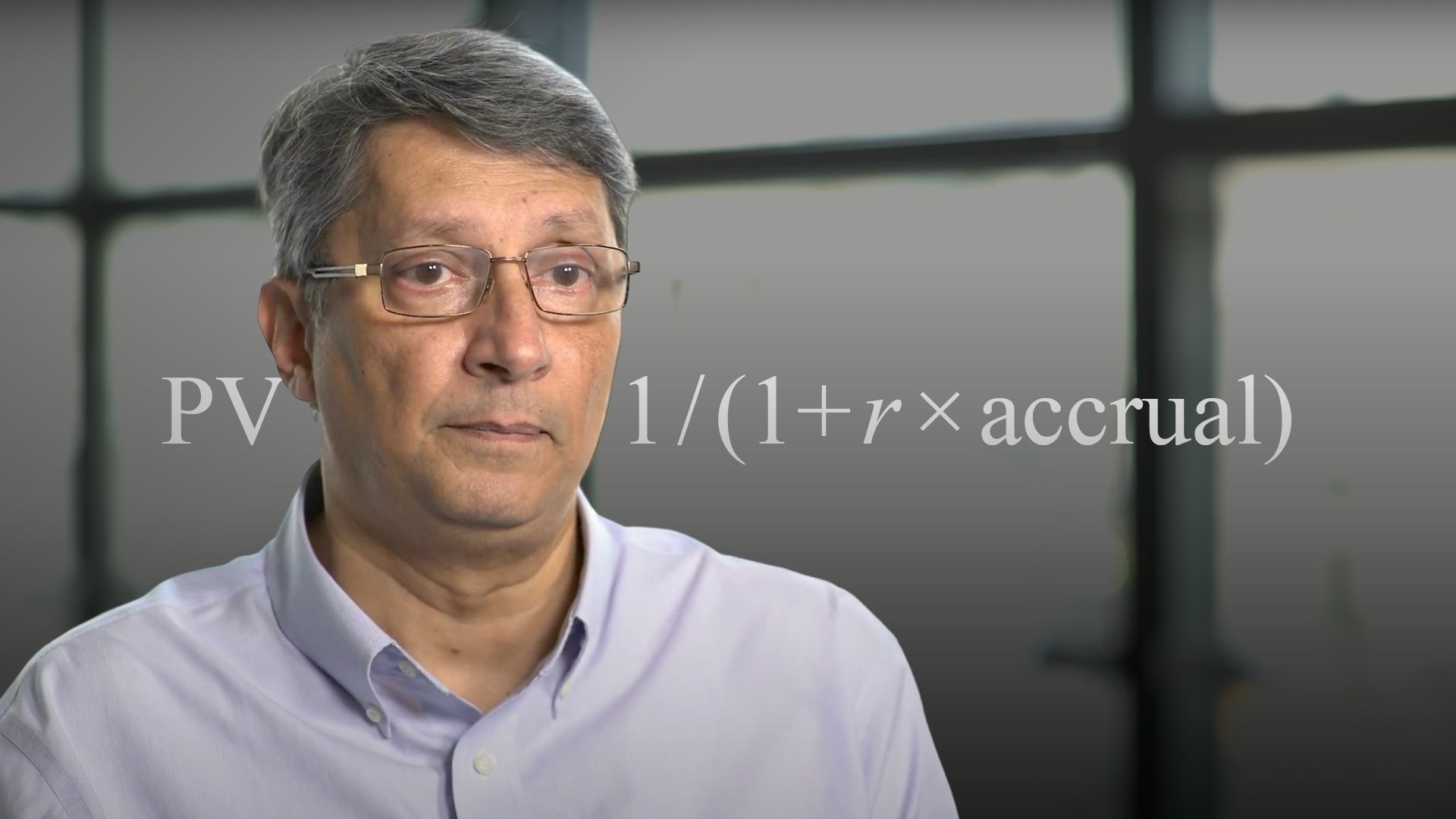03:46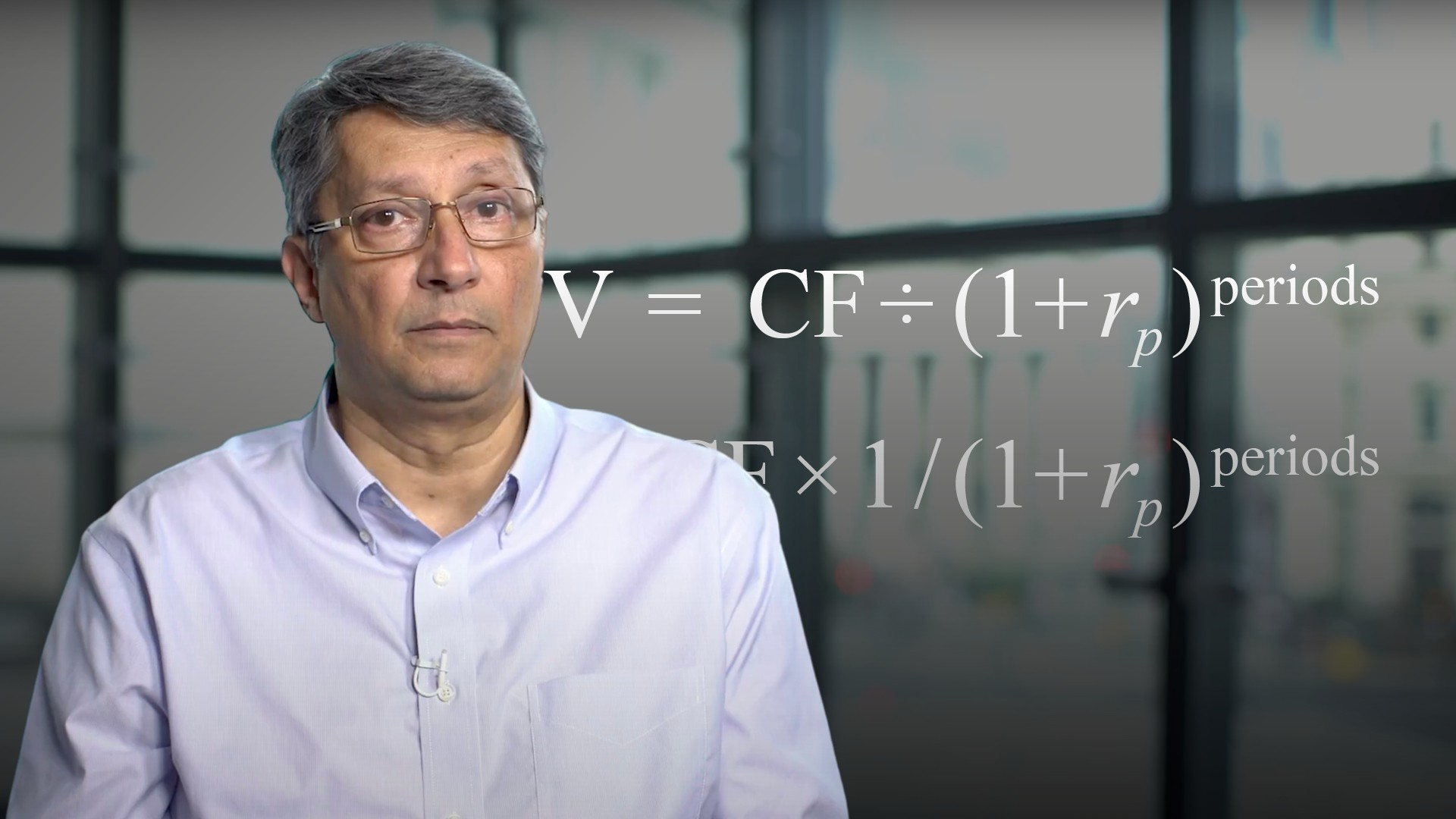04:19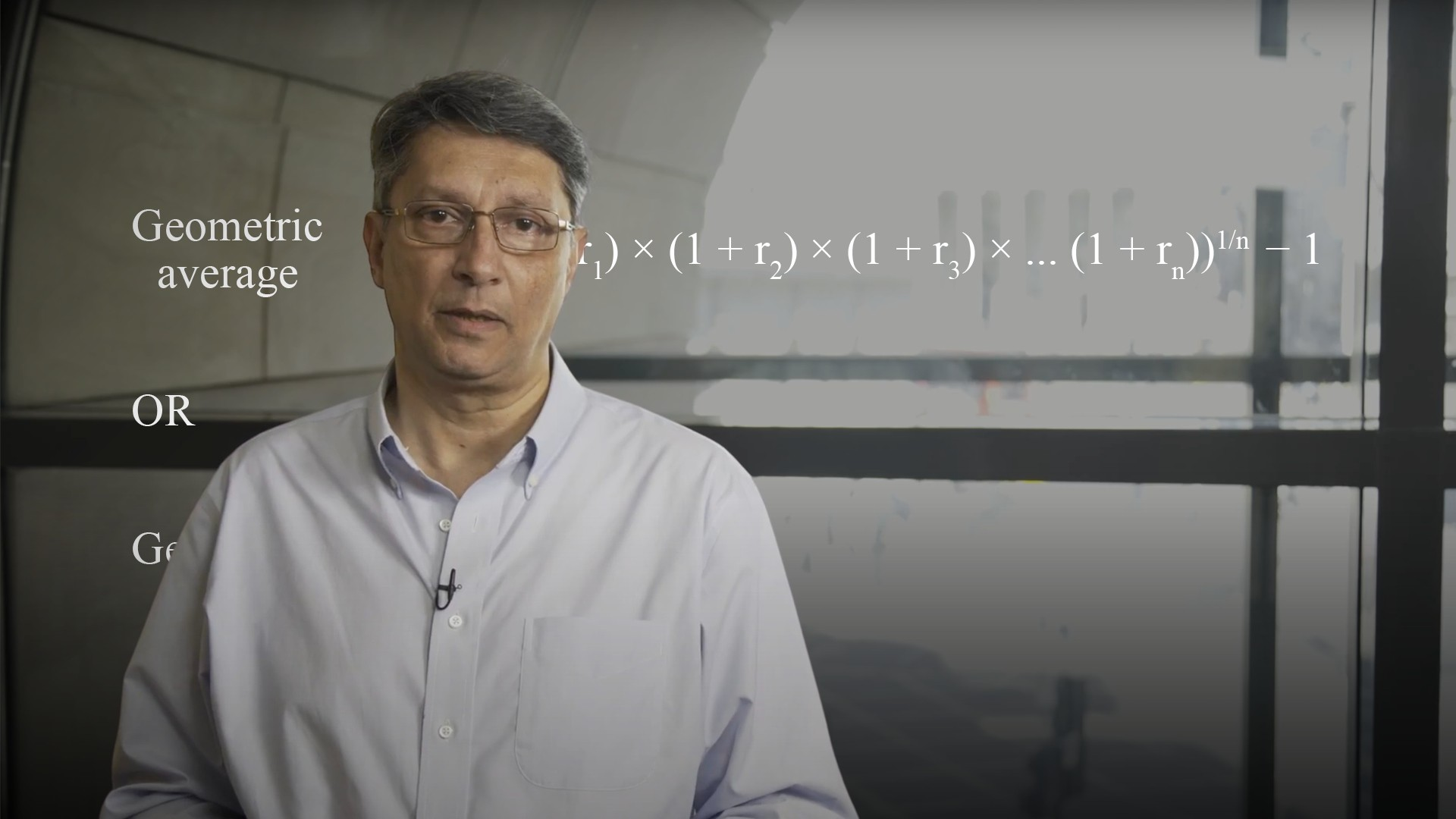04:41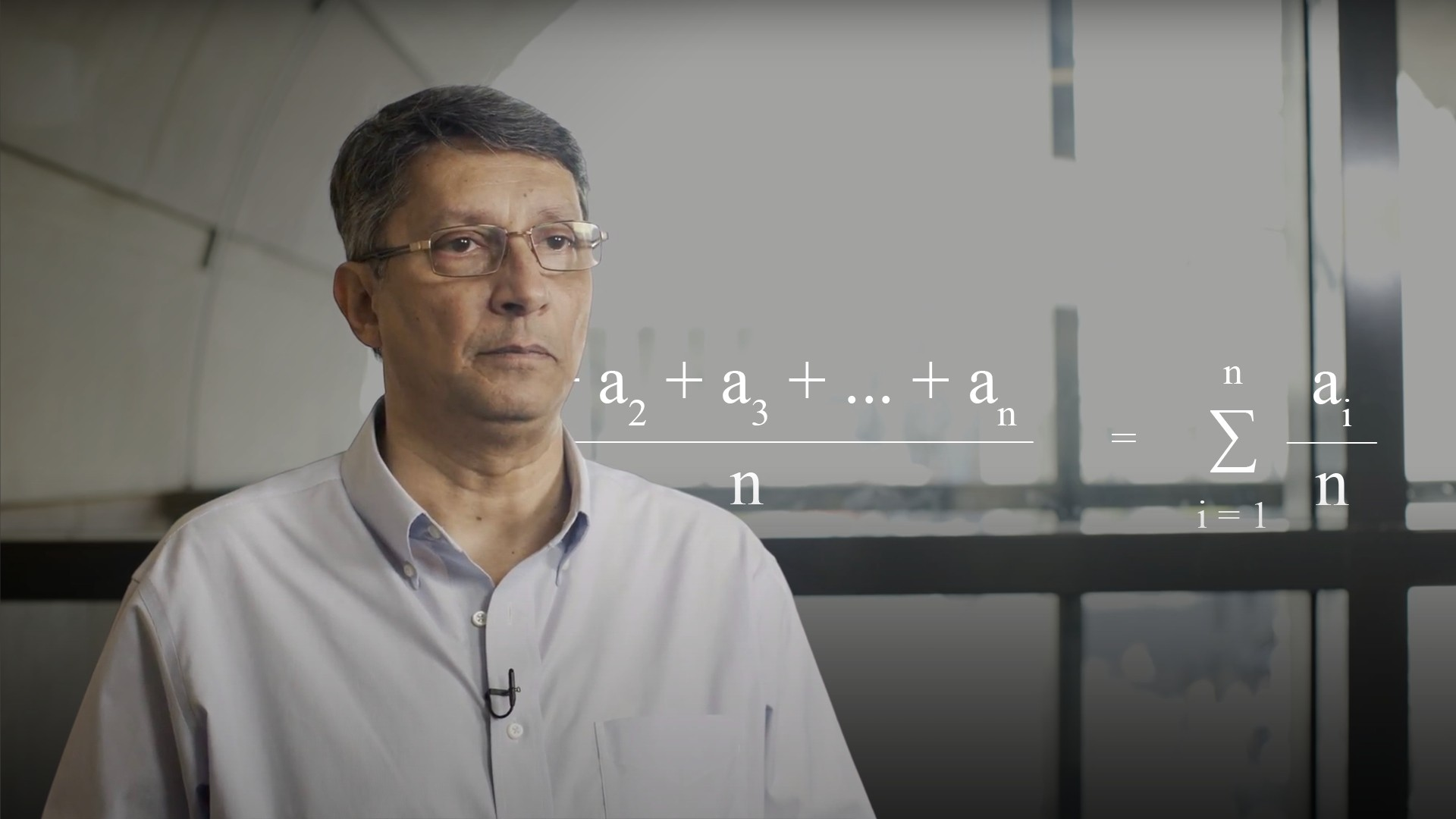04:1605:2405:39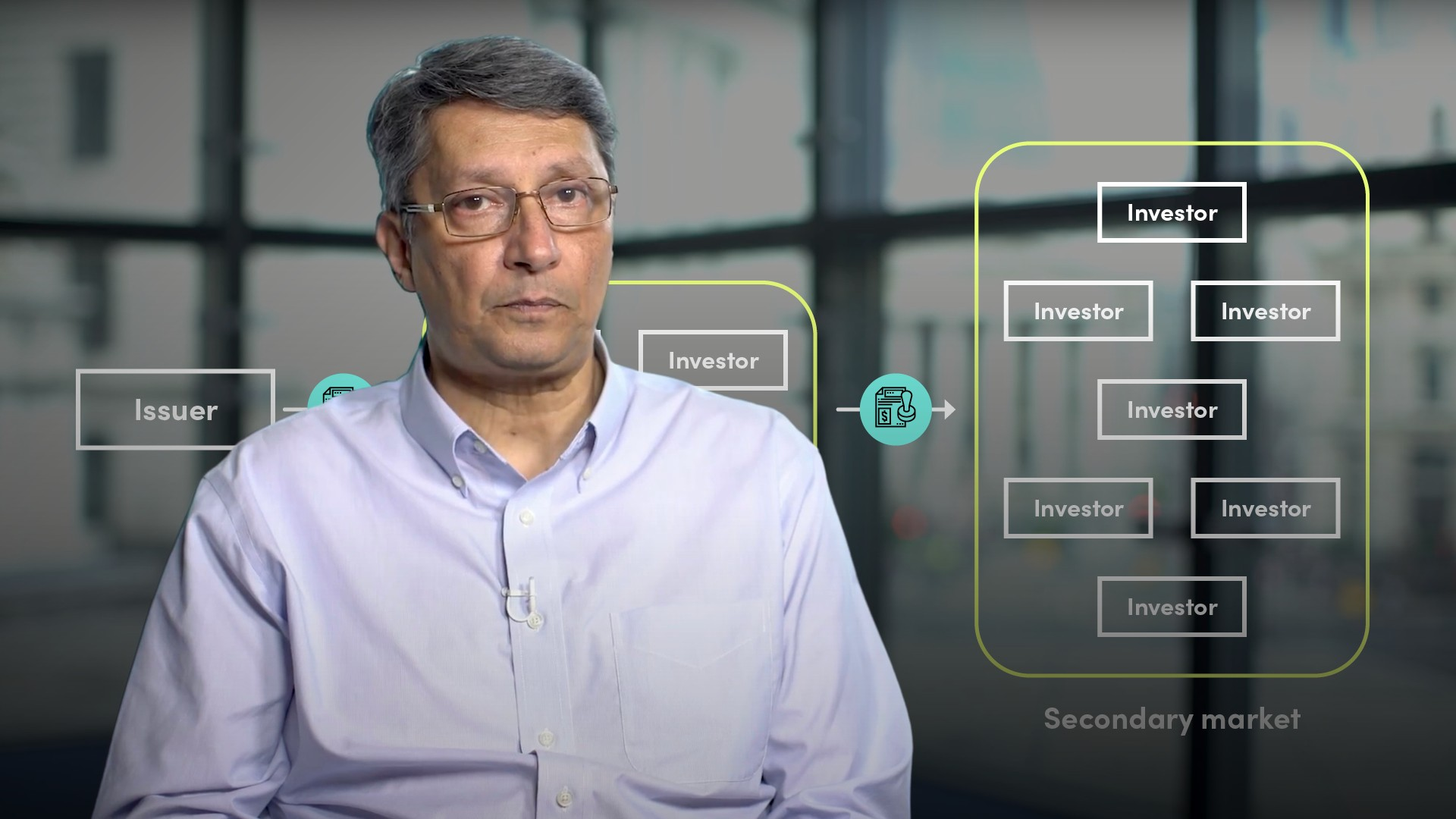06:13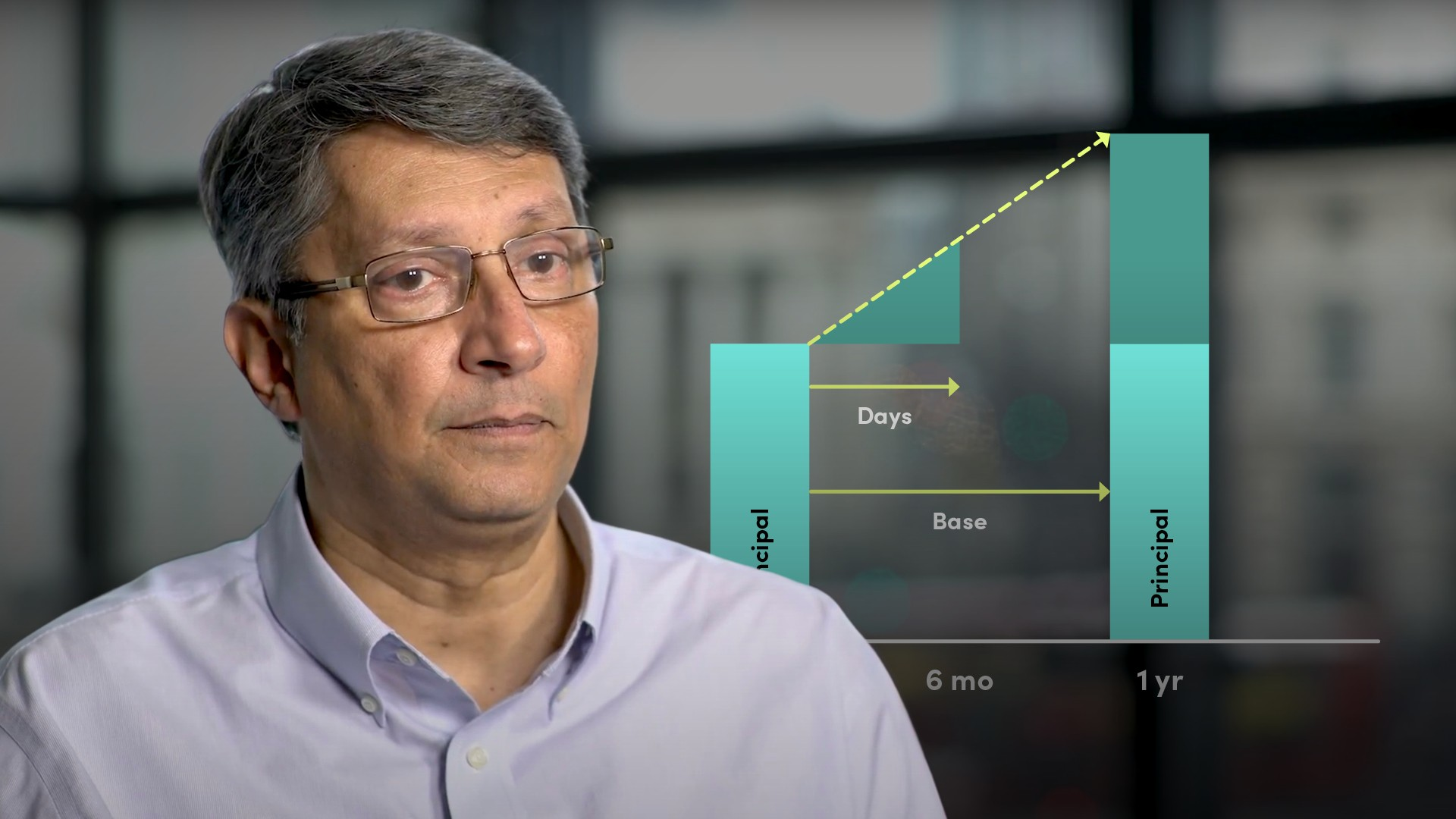05:27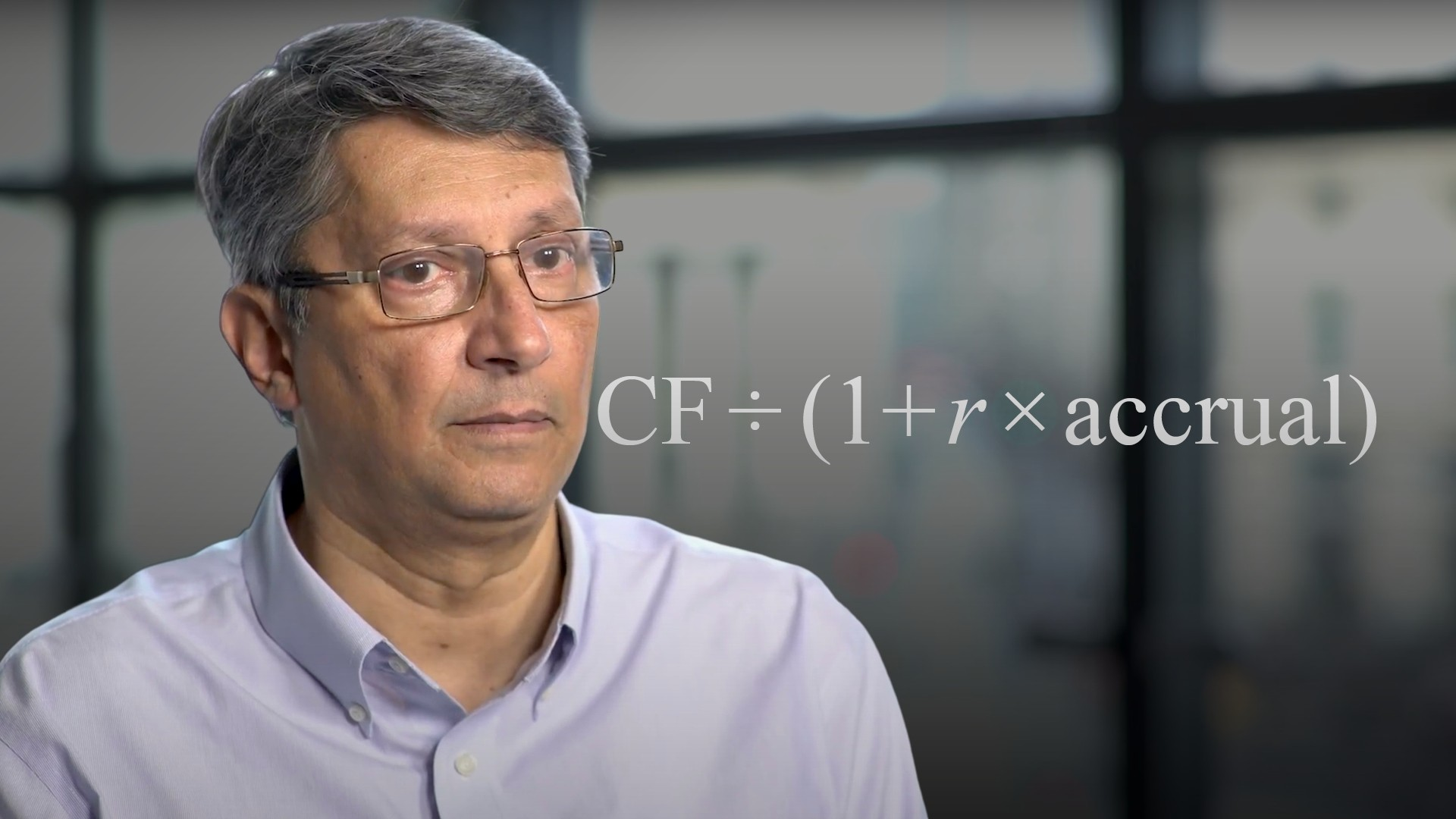03:55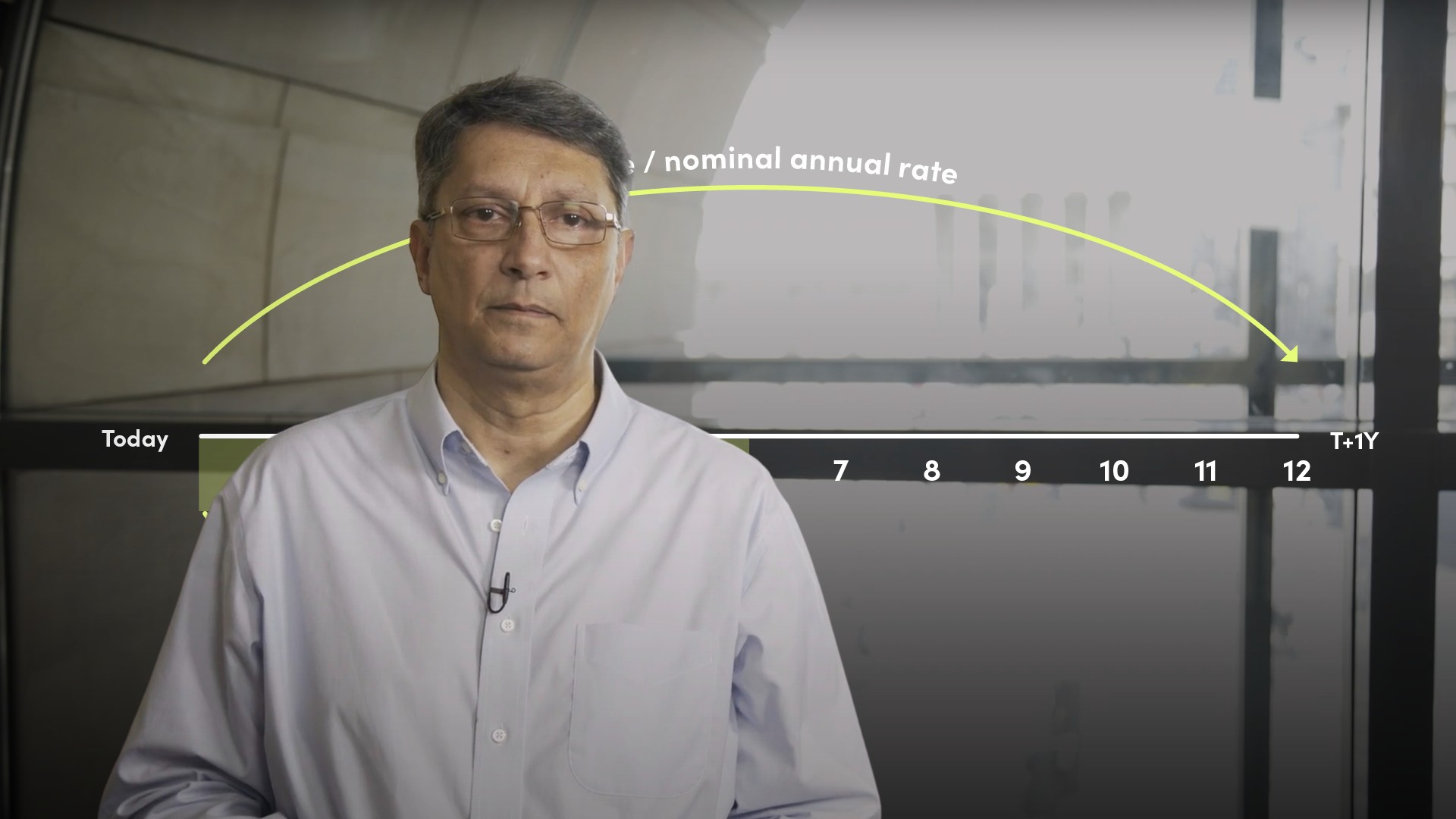07:58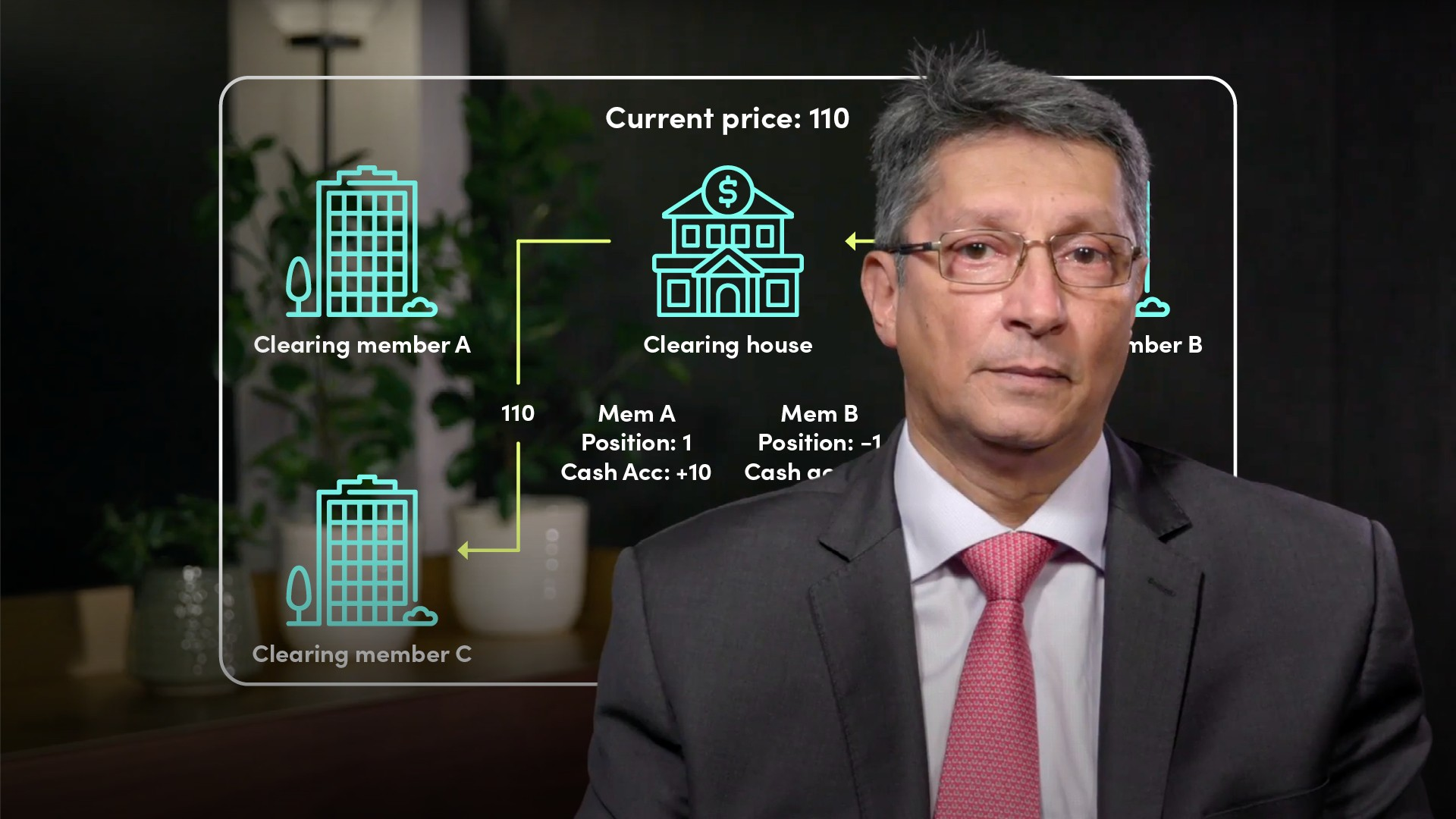05:22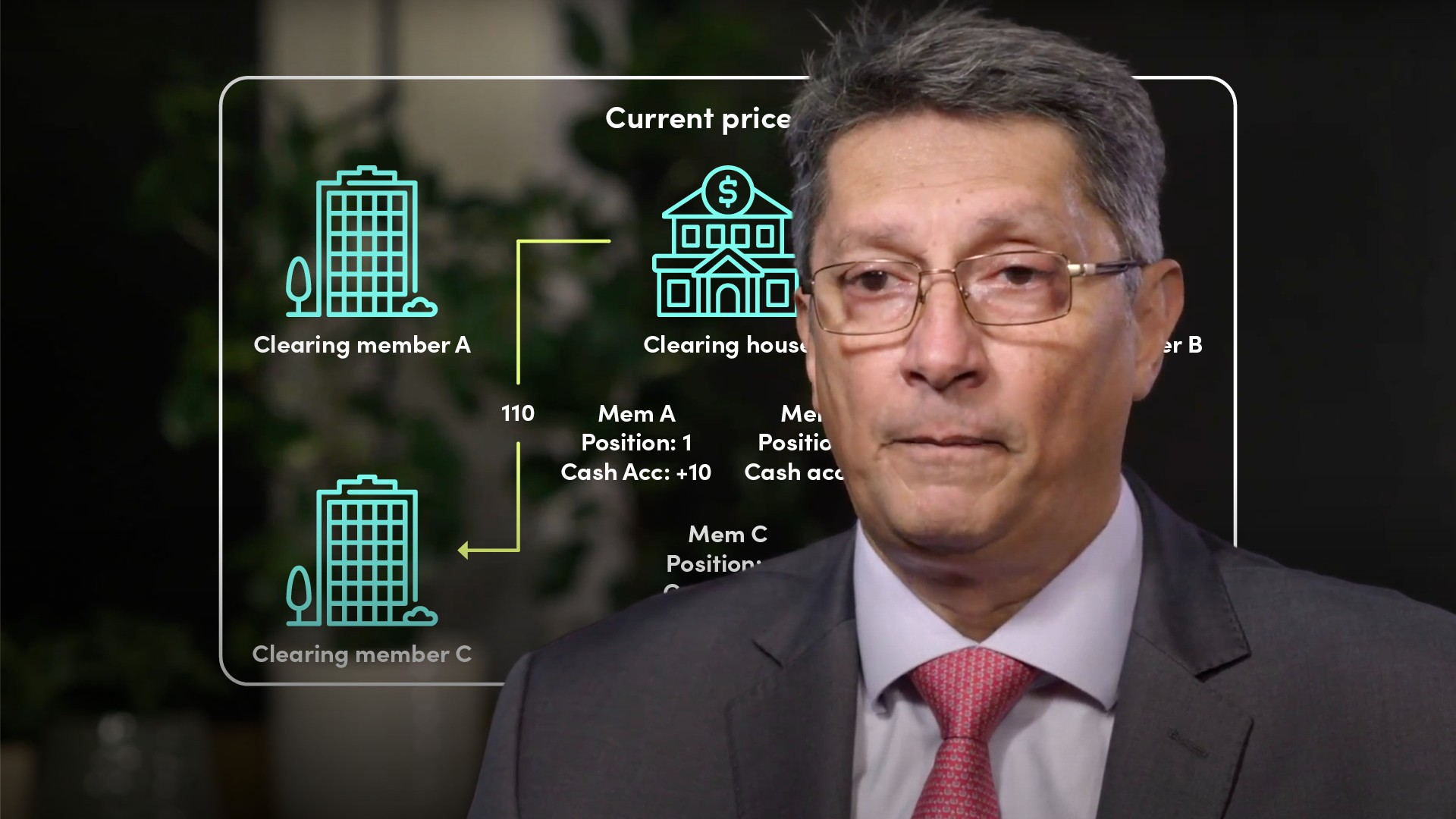05:14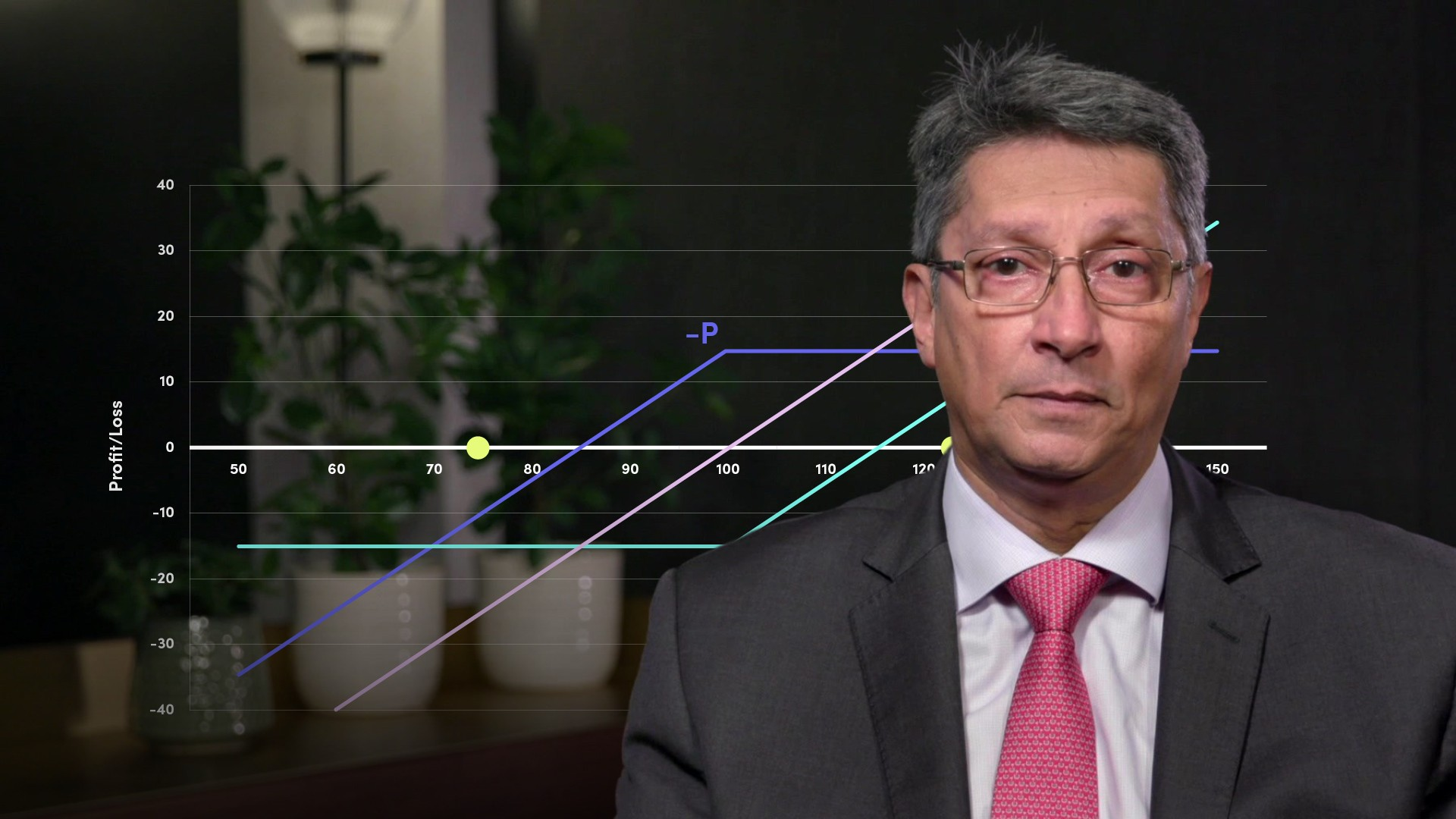06:01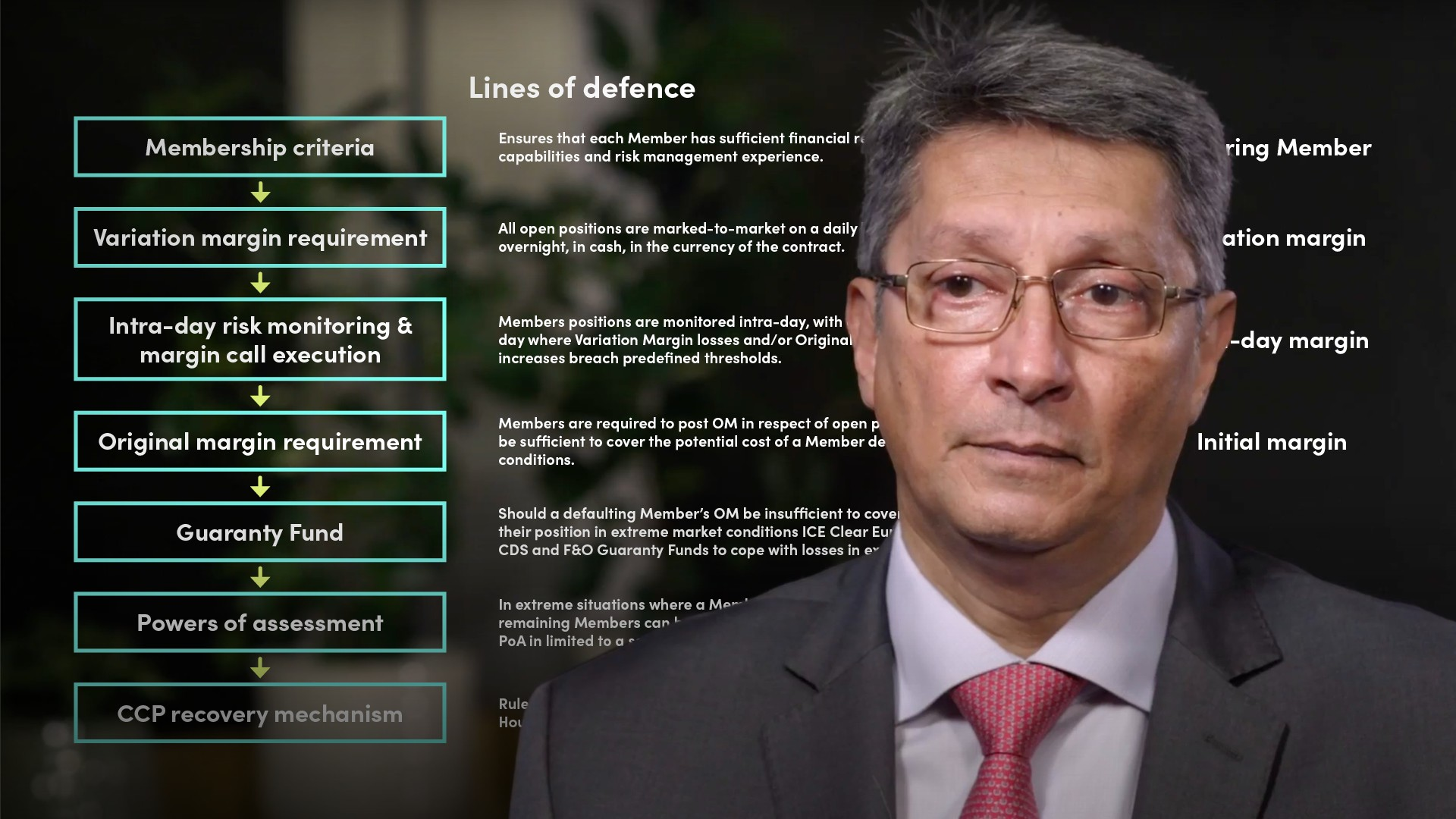06:1306:23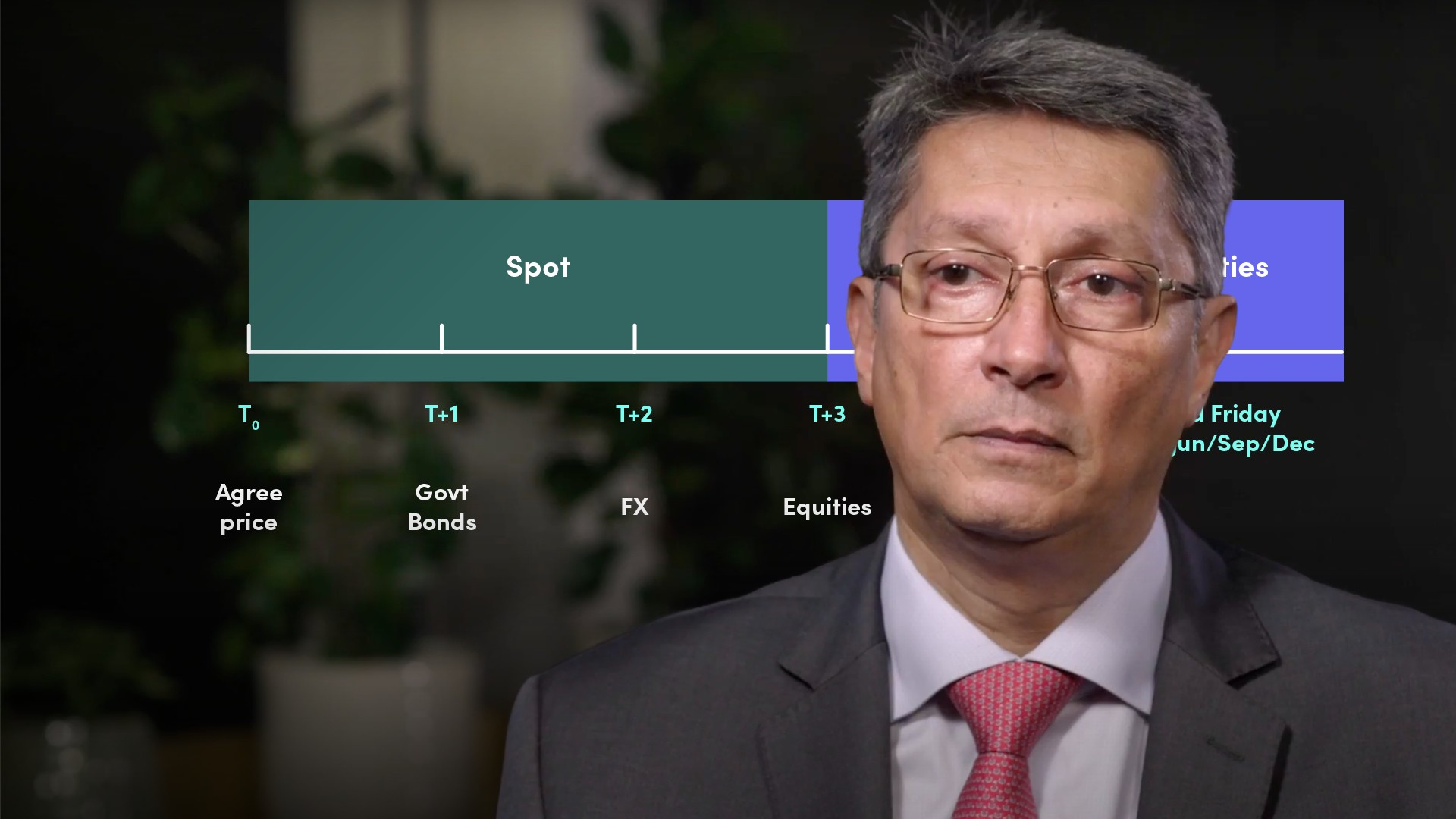05:3807:23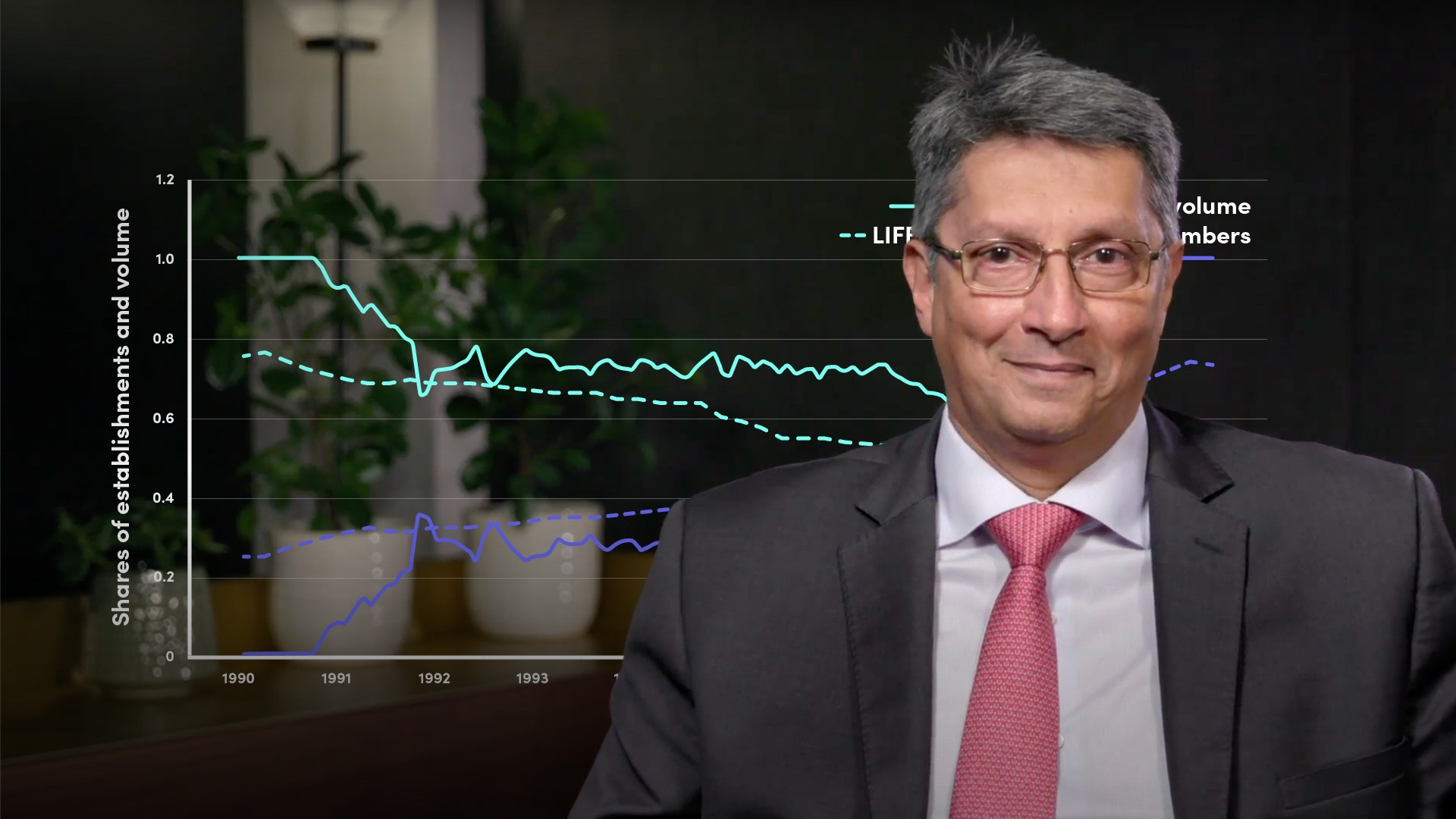04:01

## Related videosFinancial MathsFinancial MathsFinancial MathsFinancial MathsFinancial Maths### Accredited by